# JP2011050198A - Driving system of permanent magnet synchronous motor - Google Patents

## Info

Publication number
JP2011050198A
JP2011050198A JP2009197571A JP2009197571A JP2011050198A JP 2011050198 A JP2011050198 A JP 2011050198A JP 2009197571 A JP2009197571 A JP 2009197571A JP 2009197571 A JP2009197571 A JP 2009197571A JP 2011050198 A JP2011050198 A JP 2011050198A
Authority
JP
Japan
Prior art keywords
phase
current
voltage
value
synchronous motor
Prior art date
Legal status (The legal status is an assumption and is not a legal conclusion. Google has not performed a legal analysis and makes no representation as to the accuracy of the status listed.)
Granted
Application number
JP2009197571A
Other languages
Japanese (ja)
Other versions
JP5150585B2 (en
Inventor
Yoshitaka Iwaji
Daigo Kaneko
Toshibumi Sakai
Kiyoshi Sakamoto
Yoshiyuki Taguchi

Original Assignee
Hitachi Industrial Equipment Systems Co Ltd

Priority date (The priority date is an assumption and is not a legal conclusion. Google has not performed a legal analysis and makes no representation as to the accuracy of the date listed.)
Filing date
Publication date
Application filed by Hitachi Industrial Equipment Systems Co Ltd, 株式会社日立産機システム filed Critical Hitachi Industrial Equipment Systems Co Ltd
Priority to JP2009197571A priority Critical patent/JP5150585B2/en
Publication of JP2011050198A publication Critical patent/JP2011050198A/en
Application granted granted Critical
Publication of JP5150585B2 publication Critical patent/JP5150585B2/en
Active legal-status Critical Current
Anticipated expiration legal-status Critical

• 230000001360 synchronised Effects 0.000 title claims abstract description 107
• 244000171263 Ribes grossularia Species 0.000 claims abstract description 571
• 239000000969 carriers Substances 0.000 claims description 38
• 238000004804 winding Methods 0.000 claims description 21
• 230000000051 modifying Effects 0.000 claims description 9
• 230000003247 decreasing Effects 0.000 claims description 3
• 230000004907 flux Effects 0.000 description 37
• 238000010586 diagrams Methods 0.000 description 29
• 238000000034 methods Methods 0.000 description 22
• 238000004364 calculation methods Methods 0.000 description 12
• 230000000694 effects Effects 0.000 description 11
• 230000014509 gene expression Effects 0.000 description 9
• 238000006243 chemical reactions Methods 0.000 description 7
• 239000003990 capacitor Substances 0.000 description 5
• 230000001419 dependent Effects 0.000 description 5
• 101710030746 Vesicle-Associated Membrane Protein 1 Proteins 0.000 description 3
• 101710030743 Vesicle-Associated Membrane Protein 3 Proteins 0.000 description 3
• 230000000875 corresponding Effects 0.000 description 3
• 238000005070 sampling Methods 0.000 description 3
• 230000005428 wave function Effects 0.000 description 3
• 230000003313 weakening Effects 0.000 description 3
• 241000169085 Philydraceae Species 0.000 description 2
• -1 Vamp2 Proteins 0.000 description 2
• 230000001276 controlling effects Effects 0.000 description 2
• 229910003460 diamond Inorganic materials 0.000 description 2
• 239000010432 diamond Substances 0.000 description 2
• 239000000203 mixtures Substances 0.000 description 2
• 230000001131 transforming Effects 0.000 description 2
• 101710030740 VAMP4 Proteins 0.000 description 1
• 239000002131 composite materials Substances 0.000 description 1
• 238000007796 conventional methods Methods 0.000 description 1
• 238000001514 detection method Methods 0.000 description 1
• 238000002360 preparation methods Methods 0.000 description 1
• 230000000576 supplementary Effects 0.000 description 1
• 230000001629 suppression Effects 0.000 description 1
• 230000001052 transient Effects 0.000 description 1

## Images

•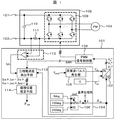•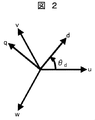••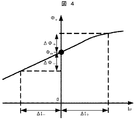••••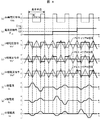•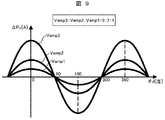•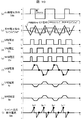•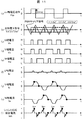•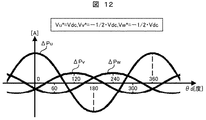•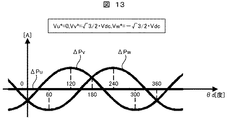•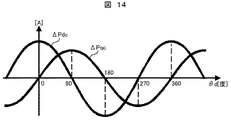•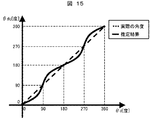•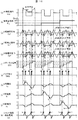•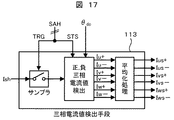•••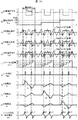•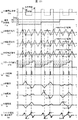•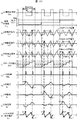•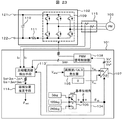•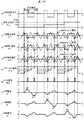•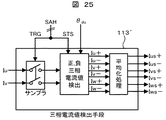•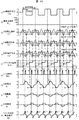•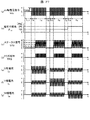•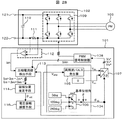••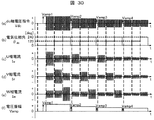•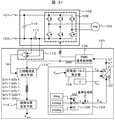•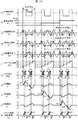•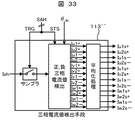•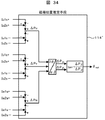•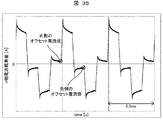••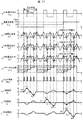•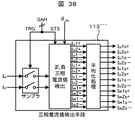## Classifications

• HELECTRICITY
• H02GENERATION; CONVERSION OR DISTRIBUTION OF ELECTRIC POWER
• H02PCONTROL OR REGULATION OF ELECTRIC MOTORS, ELECTRIC GENERATORS OR DYNAMO-ELECTRIC CONVERTERS; CONTROLLING TRANSFORMERS, REACTORS OR CHOKE COILS
• H02P9/00Arrangements for controlling electric generators for the purpose of obtaining a desired output
• H02P9/14Arrangements for controlling electric generators for the purpose of obtaining a desired output by variation of field
• H02P9/26Arrangements for controlling electric generators for the purpose of obtaining a desired output by variation of field using discharge tubes or semiconductor devices
• H02P9/30Arrangements for controlling electric generators for the purpose of obtaining a desired output by variation of field using discharge tubes or semiconductor devices using semiconductor devices
• HELECTRICITY
• H02GENERATION; CONVERSION OR DISTRIBUTION OF ELECTRIC POWER
• H02MAPPARATUS FOR CONVERSION BETWEEN AC AND AC, BETWEEN AC AND DC, OR BETWEEN DC AND DC, AND FOR USE WITH MAINS OR SIMILAR POWER SUPPLY SYSTEMS; CONVERSION OF DC OR AC INPUT POWER INTO SURGE OUTPUT POWER; CONTROL OR REGULATION THEREOF
• H02M7/00Conversion of ac power input into dc power output; Conversion of dc power input into ac power output
• H02M7/42Conversion of dc power input into ac power output without possibility of reversal
• H02M7/44Conversion of dc power input into ac power output without possibility of reversal by static converters
• H02M7/48Conversion of dc power input into ac power output without possibility of reversal by static converters using discharge tubes with control electrode or semiconductor devices with control electrode
• H02M7/53Conversion of dc power input into ac power output without possibility of reversal by static converters using discharge tubes with control electrode or semiconductor devices with control electrode using devices of a triode or transistor type requiring continuous application of a control signal
• H02M7/537Conversion of dc power input into ac power output without possibility of reversal by static converters using discharge tubes with control electrode or semiconductor devices with control electrode using devices of a triode or transistor type requiring continuous application of a control signal using semiconductor devices only, e.g. single switched pulse inverters
• H02M7/5387Conversion of dc power input into ac power output without possibility of reversal by static converters using discharge tubes with control electrode or semiconductor devices with control electrode using devices of a triode or transistor type requiring continuous application of a control signal using semiconductor devices only, e.g. single switched pulse inverters in a bridge configuration
• H02M7/53871Conversion of dc power input into ac power output without possibility of reversal by static converters using discharge tubes with control electrode or semiconductor devices with control electrode using devices of a triode or transistor type requiring continuous application of a control signal using semiconductor devices only, e.g. single switched pulse inverters in a bridge configuration with automatic control of output voltage or current
• H02M7/53873Conversion of dc power input into ac power output without possibility of reversal by static converters using discharge tubes with control electrode or semiconductor devices with control electrode using devices of a triode or transistor type requiring continuous application of a control signal using semiconductor devices only, e.g. single switched pulse inverters in a bridge configuration with automatic control of output voltage or current with digital control
• HELECTRICITY
• H02GENERATION; CONVERSION OR DISTRIBUTION OF ELECTRIC POWER
• H02PCONTROL OR REGULATION OF ELECTRIC MOTORS, ELECTRIC GENERATORS OR DYNAMO-ELECTRIC CONVERTERS; CONTROLLING TRANSFORMERS, REACTORS OR CHOKE COILS
• H02P21/00Arrangements or methods for the control of electric machines by vector control, e.g. by control of field orientation
• H02P21/14Estimation or adaptation of machine parameters, e.g. flux, current or voltage
• H02P21/18Estimation of position or speed
• HELECTRICITY
• H02GENERATION; CONVERSION OR DISTRIBUTION OF ELECTRIC POWER
• H02PCONTROL OR REGULATION OF ELECTRIC MOTORS, ELECTRIC GENERATORS OR DYNAMO-ELECTRIC CONVERTERS; CONTROLLING TRANSFORMERS, REACTORS OR CHOKE COILS
• H02P6/00Arrangements for controlling synchronous motors or other dynamo-electric motors using electronic commutation dependent on the rotor position; Electronic commutators therefor
• H02P6/14Electronic commutators
• H02P6/16Circuit arrangements for detecting position
• H02P6/18Circuit arrangements for detecting position without separate position detecting elements
• HELECTRICITY
• H02GENERATION; CONVERSION OR DISTRIBUTION OF ELECTRIC POWER
• H02PCONTROL OR REGULATION OF ELECTRIC MOTORS, ELECTRIC GENERATORS OR DYNAMO-ELECTRIC CONVERTERS; CONTROLLING TRANSFORMERS, REACTORS OR CHOKE COILS
• H02P9/00Arrangements for controlling electric generators for the purpose of obtaining a desired output
• H02P9/009Circuit arrangements for detecting rotor position

## Abstract

In a control device and a control method for realizing control of a synchronous motor, when the same high-frequency voltage command is generated on two orthogonal axes, a difference occurs in applied voltages in three phases, and a detected value of a generated pulsating current The estimation accuracy of the initial magnetic pole position estimation using is deteriorated.
When applying a positive and negative alternating harmonic voltage to a permanent magnet synchronous motor, the applied voltage phase is sequentially switched by 120 degrees and applied to three phases. The pulsating current generated by the application of the high-frequency voltage has elapsed for a predetermined time Δt from the state where the three-phase output voltage of the power converter is all positive or all negative, and at least the one-phase output voltage has changed. Detect with timing. The current is detected using a DC resistance attached to the DC bus or a phase current sensor. The magnetic pole position estimation means calculates the rotor magnetic pole position of the permanent magnet synchronous motor based on the difference between the positive change amount and the negative change amount of the three-phase current obtained from the detected current value.
[Selection] Figure 1

## Description

The present invention relates to control of a synchronous motor using a permanent magnet as a field, and more particularly to a control device and a control method for realizing control of a synchronous motor without using a sensor that detects a rotor magnetic pole position.

As a method for controlling the synchronous motor without detecting the rotor magnetic pole position, there are techniques disclosed in [Patent Document 1] and [Patent Document 2].

[Patent Document 1] gives a minute change to the voltage command on the dc axis which is an estimated magnetic pole axis of the synchronous motor and the qc axis orthogonal thereto, and as a result, the positive side of the current pulsation component appearing on the DC bus The rotor magnetic pole position of the synchronous motor is directly estimated using the difference between the current value and the negative current value, or the difference in current change rate between the positive and negative sides of the current pulsation component.

The method of [Patent Document 1] uses the magnetic saturation characteristics of the iron core for magnetic pole position estimation. In general, in a synchronous motor, when a high-frequency alternating voltage is applied on the d-axis, which is the direction of the rotor magnetic flux (permanent magnet magnetic flux), the magnetic flux is saturated and the inductance decreases when a current flows in the direction of increasing the magnet magnetic flux. Therefore, the current change amount (ΔI +) increases. On the contrary, when the current flows in the direction of weakening the magnetic flux of the magnet, the magnetic flux decreases and the inductance increases or becomes constant. Therefore, the current change amount (ΔI−) becomes smaller than the aforementioned ΔI +. As a result, when an alternating voltage that changes between positive and negative is injected on the d-axis, an asymmetric current flows between positive and negative.

[Patent Document 1] applies an alternating voltage to two orthogonal phase angles, and observes the asymmetric current characteristics described above from the detected DC bus current. Since the rotor magnetic pole position is unknown, an alternating voltage is applied at an arbitrary phase angle, but the asymmetric current characteristics generated at that time vary depending on the rotor magnetic pole position. [Patent Document 1] calculates the rotor magnetic pole position using an arc tangent function on the assumption that the asymmetric current characteristic changes with the sin function and the cos function of the rotor magnetic pole position. According to the method of [Patent Document 1], the rotor magnetic pole position can be accurately estimated without being affected by the difference in structure of the synchronous motor such as salient poles and non-salient poles.

The method of [Patent Document 2] also uses the magnetic saturation characteristics of the iron core for estimating the rotor magnetic pole position. [Patent Document 2] applies a positive and negative alternating voltage to each of the three-phase stator winding shafts, and detects a phase current on the shaft to which the alternating voltage is applied. When the positive phase current detection values for three phases are obtained, the magnitudes of the phases are compared to search for the phase having the maximum value, and the rotor magnetic pole position is determined with a resolution of 120 degrees. Further, in order to determine the rotor magnetic pole position in more detail, a current difference magnification α, which will be described later, is calculated from the measured positive phase current detection value for the three phases, and the characteristic data (rotor is measured in advance). Comparison with position and magnification α).

In addition, it is disclosed that the magnification α is calculated by the following expression when the positive phase current detection value is the maximum current, intermediate current, and minimum current. According to the method of [Patent Document 2], it is not necessary to prepare a large number of complicated arithmetic expressions in advance, and the detection of the rotor position can be easily and accurately estimated.

JP 2006-158101 A JP 2007-174721 A

In [Patent Document 1], an alternating voltage command having the same amplitude value is created for two orthogonal axes (the dc axis that is an estimated axis of the magnetic flux inside the synchronous motor and the qc axis that is orthogonal to the dc axis). However, even if the alternating voltage command values on the dc axis and the qc axis have the same amplitude, the pulse voltage of each phase is greatly different when viewed from the three-phase voltage pulse after pulse width modulation. As a result, the magnitude of the voltage applied to the u, v, and w phase stator windings changes, and the influence of magnetic saturation corresponding to the magnitude of the applied voltage appears in the detected current of each phase. Therefore, the magnetic pole position estimation method of [Patent Document 1] has a problem that the position estimation error becomes large because the three-phase current detected from the shunt resistance when the two orthogonal axes are applied does not have the magnetic saturation characteristics of the same condition.

[Patent Document 1] discloses a sample timing for detecting a shunt current attached to a DC bus. However, in the disclosed method, the V-phase current (or W-phase current) that can be detected when the qc-axis voltage is applied is the positive peak value and the negative peak value due to the limitation of detecting the shunt current attached to the DC bus. Only one, not both. Furthermore, in [Patent Document 1], the current change amount at the time of applying the qc-axis voltage is calculated, but the calculation method is established under the assumption that the influences of magnetic saturation of the V phase and the W phase are equal. . Actually, since the number of flux linkages is different between the V phase and the W phase, the influence of magnetic saturation is not equal, and the disclosed current change calculation method is not accurate. As a result, if the magnetic pole position of the synchronous motor is estimated by the method of [Patent Document 1], there is a problem that the estimation error becomes large.

In [Patent Document 2], the rotor position is determined in detail with reference to magnetically saturated current characteristic data. For this reason, it is necessary to grasp in advance the current characteristics of the electric motor to be driven. In addition, since the current characteristics change when the motor to be driven is changed, it is considered that it is necessary to review the amplitude of the alternating voltage to be applied and the discriminant value used for determining the rotor position each time. Necessary.

Also, in [Patent Document 2], in order to increase the position estimation accuracy, it is necessary to increase the number of discriminating values by referring to the magnification α of the previous operation and the current characteristics. Increasing the discriminant value is meant to make the judgment range finer, and it is likely that erroneous determination of magnetic pole position discrimination is likely to occur due to the influence of noise or the like. In order to reduce the erroneous determination, it is necessary to apply a high voltage and increase the motor current so as to cause the magnetic saturation more strongly, thereby widening the boundary between the determination values. However, as a result, there is a problem that noise generated from the motor is increased.

In view of the above problems, an object of the present invention is to provide a power converter control method and current detection means that make the magnetic saturation condition equal to the three-phase detection current value used for position estimation calculation. There is. As another object, a synchronous motor that can accurately estimate the magnetic pole position from the three-phase detection current without knowing the current characteristics of the motor to be driven in advance and adjusting the discriminant value. The present invention provides a control device and a control method.

In order to achieve the above object, the present invention provides a synchronous motor driving apparatus that alternates between positive and negative voltage application conditions in order to equalize the three-phase detection current values used for position estimation calculation. When applying the harmonic voltage to be applied, the applied voltage phase is sequentially switched by 120 degrees and applied to the three phases. A positive current value and a negative current value of the phase current generated by applying each harmonic voltage are detected from the DC bus current, and a deviation amount between the positive and negative current values of each of the three phases is calculated. The deviation amount of the three phases is coordinate-converted into two orthogonal axes, and the rotor magnetic pole position is calculated using arc tangent.

Specifically, in order to achieve the above object, the present invention relates to a synchronous motor, a PWM signal control unit that performs pulse width modulation of a three-phase voltage command signal using a carrier signal, and power driven by a pulse signal that is pulse width modulated. A synchronous motor driving device comprising: a converter; command voltage generating means for generating a three-phase voltage command signal for applying a high-frequency voltage to the synchronous motor; and the synchronous motor when the high-frequency voltage is applied to the synchronous motor. Current detection means for detecting a flowing current, and magnetic pole position estimation means for estimating the magnetic pole position of the synchronous motor based on a current detection result, wherein the command voltage generation means is positive in an arbitrary phase with an arbitrary voltage amplitude. A first applied voltage mode for applying a negatively alternating harmonic voltage, and a second for applying a high-frequency voltage having the same amplitude to a phase shifted by 120 degrees in electrical angle from the phase. A three-phase voltage command signal is generated by sequentially switching between the applied voltage mode and a third applied voltage mode for applying a high-frequency voltage having the same amplitude to a phase shifted by 240 degrees in electrical angle from the phase. The positive side value and the negative side value of the pulsating current generated by the application of the high frequency voltage in the second and third applied voltage modes are detected one by one in each mode, the positive U phase detection current value, the negative side value Information on the U-phase detection current value, positive-side V-phase detection current value, negative-side V-phase detection value, positive-side W-phase detection current value, and negative-side W-phase detection current value is obtained to estimate the magnetic pole position. The means calculates the estimated magnetic pole position of the synchronous motor using the detected current value of each phase on the positive side and the detected current value of each phase on the negative side.

Furthermore, in the synchronous motor drive device according to the present invention, the command voltage generating means sets the phase direction of the phase θ from the reference phase with the winding axis direction of an arbitrary phase of the three-phase stator winding of the synchronous motor as a reference phase. = 0.

Further, in the synchronous motor driving device according to the present invention, the three-phase voltage command signal generated by the command voltage generating means has a minimum pattern of applied voltage in which two periods of the carrier signal of the PWM signal control unit are one unit. It is characterized by being repeated one or more times.

Furthermore, in the synchronous motor drive device according to the present invention, the minimum pattern holds at least one of the three-phase voltage commands positive during a half cycle of the carrier signal, and the voltage commands of the remaining phases. Is negative, and the polarity of the three-phase voltage command is reversed for one period of the subsequent carrier signal, and the polarity of the three-phase voltage command is reversed again for the half period of the subsequent carrier signal. It is a feature.

Further, in the synchronous motor driving device according to the present invention, the command voltage generating means outputs a special three-phase voltage command before releasing the all-phase off state of the gate signal and applying the high-frequency voltage, A period for turning on all lower arm switch elements of the power converter is provided.

Furthermore, in the synchronous motor driving device according to the present invention, when the applied voltage mode is sequentially switched, the command voltage generating means sets all the three-phase voltage commands to zero and provides a period during which the application of the high-frequency voltage is suspended. It is a feature.

Furthermore, in the synchronous motor driving apparatus, the present invention includes an averaging processing means for averaging current values detected at least one point in each of the first, second, and third applied voltage modes for each detection point. , Positive U phase detection current average value, negative U phase detection current average value, positive V phase detection current average value, negative V phase detection average value, positive W phase detection current average value, Obtaining information on the negative-side W-phase detected current average value, and using the detected current average value of each positive-side phase and the detected current average value of each negative-side phase, the estimated magnetic pole of the synchronous motor The position is calculated.

Further, in the synchronous motor driving device according to the present invention, the average value of the detected current is a current after a predetermined time has elapsed since the application of the high-frequency voltage in the first, second, and third applied voltage modes. It is calculated using a detected value.

Furthermore, the present invention is a synchronous motor drive device, wherein the current detection means detects a DC bus current of the power converter.

Furthermore, in the synchronous motor drive device according to the present invention, the current detection unit is configured to change the output voltage of at least one phase from a state in which all three-phase output voltages of the power converter are all positive or all negative. Further, the DC bus current is detected at a timing when a predetermined time Δt has elapsed.

Furthermore, in the synchronous motor drive device according to the present invention, the current detection unit is configured to change the output voltage of at least one phase from a state in which all three-phase output voltages of the power converter are all positive or all negative. From the time when the predetermined time Δt has elapsed and the state in which the three-phase output voltages of the power converter are all positive or all negative, the predetermined time Kt × Δt from the time when the output voltage of at least one phase has changed. The DC bus current is detected at a timing when only a lapse of time (Kt is a positive fixed value of 1 or less).

Furthermore, in the synchronous motor drive device according to the present invention, the current detection means is a timing at which the three-phase output voltages of the power converter transition to a state in which all are positive (maximum value) or all are negative (minimum value). The DC bus current is detected in the vicinity of.

Furthermore, in the synchronous motor drive device according to the present invention, the current detection means is at least one from a state in which the three-phase output voltages of the power converter are all positive (maximum value) or all negative (minimum value). It is characterized in that the DC bus current is detected in close proximity to the timing at which the phase output voltage changes and the timing to transition to a state where all are positive (maximum value) or all are negative (minimum value).

Furthermore, the present invention is characterized in that, in the synchronous motor drive device, the current detection means detects phase currents of at least two phases.

Furthermore, in the synchronous motor drive device according to the present invention, the current detection unit detects a phase current at a timing of a peak or valley of a carrier signal of the PWM signal control unit.

Furthermore, the present invention is characterized in that in the synchronous motor drive device, the current detection means detects the phase current at the timing of peaks and valleys of the carrier signal of the PWM signal control unit.

Furthermore, in the synchronous motor driving device according to the present invention, the magnetic pole position estimating means obtains a deviation amount between a positive current detection value and a negative current detection value for each phase, and the obtained deviation for the three phases is obtained. The quantity is coordinate-transformed into two orthogonal axes, and the estimated magnetic pole position of the synchronous motor is calculated from the orthogonal two-axis quantity using an arc tangent.

Further, in the synchronous motor drive device according to the present invention, the magnetic pole position estimation means obtains a positive current change amount and a negative current change amount for each phase from the current detection value, and the positive current change amount The amount of deviation between the amount and the negative current change amount is obtained for each phase, and the obtained amount of deviation for the three phases is coordinate-transformed into two orthogonal axes, and the synchronous tangent is calculated using the arctangent from the two orthogonal axes. The estimated magnetic pole position of the electric motor is calculated.

Furthermore, the present invention provides a synchronous motor drive device, comprising: an evaluation unit that calculates a predetermined evaluation value based on a magnetic pole position estimation result of the synchronous motor; and a unit that varies an amplitude of a high-frequency voltage applied to the synchronous motor, Based on the evaluation value, the amplitude of the high-frequency voltage is automatically adjusted by increasing / decreasing the amplitude.

Further, in the synchronous motor driving device according to the present invention, the evaluation value is obtained by performing the initial position estimation two or more times, and calculating the maximum value and the minimum value from the magnetic pole position estimation results for the number of times. And a deviation amount of the minimum value.

According to the present invention, by estimating the initial position by extracting the current characteristics depending on the permanent magnet magnetic pole position having the same amplitude in each of the three phases, there is an effect of improving the estimation accuracy. By improving the accuracy, the present invention has an effect of improving the starting torque of the motor to be driven.

In addition, the current characteristics of the magnetic saturation of the iron core by permanent magnets are used more strictly for initial position estimation than in the conventional method, so the minimum motor current required to satisfy the required value of estimation accuracy can be increased. Can be small. As a result, noise due to generation of pulsating current at the time of initial position estimation can be reduced, and there is an effect of noise reduction.

1 is an overall control block diagram according to a first embodiment of the present invention. The relationship diagram of the rotor magnetic pole position of a three-phase stator winding shaft and a permanent magnet synchronous motor. The relationship figure of the phase axis which applies a harmonic voltage to a three-phase stator winding axis and a permanent magnet synchronous motor. The relationship figure of synthetic | combination magnetic flux (PHI) u and U-phase electric current Iu when d-axis and u-axis correspond. The relationship figure of synthetic | combination magnetic flux (PHI) u and U-phase electric current Iu when d-axis and u-axis are orthogonal. FIG. 6 is a relationship diagram between positive and negative current changes and rotor magnetic pole positions when a harmonic voltage is applied to the u-axis. FIG. 6 is a relationship diagram between a deviation amount ΔPu of current change on the positive side and negative side and a rotor magnetic pole position when a harmonic voltage is applied to the u-axis. FIG. 6 is an operation waveform diagram when harmonic voltages are sequentially applied to the three-phase stator winding in the first embodiment of the present invention. The relationship figure of voltage amplitude instruction | command Vamp and deviation amount (DELTA) Pu of a current pulsation component. The operation waveform diagram when applying a harmonic voltage to the dc axis in the prior art. The operation waveform figure at the time of applying a harmonic voltage to qc axis in a prior art. FIG. 6 is a diagram showing the relationship between the deviation amount of positive and negative current changes flowing through a three-phase stator winding and the rotor magnetic pole position when a harmonic voltage is applied to the dc axis in the prior art. FIG. 6 is a diagram showing the relationship between the deviation amount of positive and negative current changes flowing through a three-phase stator winding and the rotor magnetic pole position when a harmonic voltage is applied to the qc axis in the prior art. FIG. 6 is a relationship diagram between a combined current deviation amount ΔPdc when a dc axis pulse voltage is applied and a combined current deviation amount ΔPqc when a qc axis pulse voltage is applied in the prior art. The example of the estimation error which arises by orthogonal 2-axis pulse voltage application of a prior art. The example of the electric current detection timing in the 1st Embodiment of this invention. The functional block diagram of the three-phase current detection means in 1st Embodiment of this invention. The functional block diagram of the magnetic pole position estimation means in 1st Embodiment of this invention. The relationship diagram of the current deviation amount and rotor magnetic pole position in a two-phase fixed shaft orthogonal to. The another example of the electric current detection timing in the 1st Embodiment of this invention. In the 1st Embodiment of this invention, the example of the electric current detection timing at the time of setting reference | standard phase angle (theta) = 15 degree | times. In the 1st Embodiment of this invention, another example of the electric current detection timing at the time of setting reference | standard phase angle (theta) = 15 degree | times. The whole control block diagram in the 2nd Embodiment of this invention. The example of the electric current detection timing in the 2nd Embodiment of this invention. The functional block diagram of the three-phase current detection means in the 2nd Embodiment of this invention. The operation | movement waveform diagram when the basic unit of a high frequency applied voltage is repeated in multiple times. The output signal suitable when using this invention actually, and its operation | movement waveform diagram. The whole control block diagram in the 3rd Embodiment of this invention. The flowchart figure which showed the voltage amplitude adjustment procedure in the 3rd Embodiment of this invention. The example of an operation | movement waveform of the voltage amplitude adjustment means in the 3rd Embodiment of this invention. The whole control block diagram in the 4th Embodiment of this invention. The example of the electric current detection timing in the 4th Embodiment of this invention. The functional block diagram of the three-phase current detection means in 4th Embodiment of this invention. The functional block diagram of the magnetic pole position estimation means in 4th Embodiment of this invention. Example of actual current waveform when positive and negative alternating voltage is applied. The whole control block diagram in the 5th Embodiment of this invention. The example of the electric current detection timing in the 5th Embodiment of this invention. The functional block diagram of the three-phase current detection means in the 5th Embodiment of this invention.

Hereinafter, embodiments of the present invention will be described in detail with reference to the drawings. In addition, about the same element, the same code | symbol is attached | subjected in principle in all the figures.

FIG. 1 is a configuration diagram of a driving apparatus for a permanent magnet synchronous motor according to a first embodiment of the present invention. This apparatus includes a permanent magnet synchronous motor 103 to be controlled, a power converter 102 that drives the permanent magnet synchronous motor 103, and a control apparatus 101 that controls the power converter 102 and the permanent magnet synchronous motor 103. The control device 101 has a dc axis that is a phase axis for applying a harmonic voltage to the permanent magnet synchronous motor 103 and a qc axis that is orthogonal to the dc axis, and gives a voltage command value of a high frequency component on the dc-qc axis. And means for detecting a current flowing through the DC resistor 111 of the power converter 102. For the pulsating component included in the detected current value, the magnetic pole position of the permanent magnet synchronous motor 103 is estimated based on the difference between the positive change amount and the negative change amount of the phase current value obtained from the pulsating component. .

The configuration diagram of FIG. 1 will be described in more detail. In FIG. 1, a command voltage generation unit 104 outputs a voltage command Vdc, a voltage command Vqc, and an electrical phase angle θdc. The voltage command Vdc is output from the high frequency pulse generator 105. On the other hand, the voltage command Vqc is set to a zero value. The high frequency pulse generator 105 receives the voltage amplitude command signal Vamp and outputs a square wave signal having an amplitude of Vamp. The electrical phase angle θdc is a signal obtained by adding the output value of the switch 106 to the reference phase angle θ (fixed value). The output of the switch 106 is selected as 0 degrees, 120 degrees, or 240 degrees. The voltage command Vdc and the voltage command Vqc are converted into voltage commands Vu * , Vv * , and Vw * on the three-phase AC axis by the dq coordinate inverse converter 107 based on the electrical phase angle θdc. The voltage commands Vu * , Vv * , Vw * are converted into a PWM pulse signal for driving the power converter 102 by a pulse width modulation (PWM) signal controller 108. The switch elements constituting the main circuit unit 109 of the power converter 102 are turned on and off in response to the PWM pulse signal from the PWM signal controller 108. As a result of controlling the switch element, a voltage for driving the permanent magnet synchronous motor 103 is output to the AC terminal of the power converter 102. The power converter 102 includes a main circuit unit 109, a DC power supply unit 110, input terminals 121 and 122 for inputting AC or DC power for driving the permanent magnet synchronous motor 103, and a DC resistor 111 for current detection. Is done. The voltage across the DC resistor 111 is amplified by the amplifier 112 and output as a DC current detection current signal Ish. The three-phase current value detection means 113 uses the detection current signal Ish, the current detection timing setting signal SAH output from the PWM signal controller 108, and the electrical phase angle θdc, and the positive detection value of the three-phase current value, and Outputs the negative detection value of the three-phase current value. The values output by the three-phase current value detection means 113 are the U-phase positive detection value Ius +, the U-phase negative detection value Ius-, the V-phase positive detection value Ivs +, the V-phase negative detection value Ivs-, and the W-phase positive. Side detection value Iws + and V-phase negative detection value Iws−. The current detection timing setting signal SAH is a composite signal, and includes a trigger signal TRG that determines the detection timing of the detection current signal Ish, and an STS signal that indicates the state of the high-frequency pulse signal to be applied. Using the Ius +, Ius−, Ivs +, Ivs−, Iws +, and Iws− output from the three-phase current value detection unit 13, the magnetic pole position estimation unit 114 calculates the estimated magnetic pole position of the permanent magnet synchronous motor 103, and θest Output.

Next, the operation of estimating the initial magnetic pole position in this embodiment will be described.

First, a coordinate axis is defined using FIG. 2 and FIG. In this embodiment, as shown in FIG. 2, the U phase of the three-phase stator winding of the permanent magnet synchronous motor 103 is the u axis, the V phase is the v axis, and the W phase is the w axis. In addition, the rotor magnetic flux direction of the permanent magnet synchronous motor 103 is d-axis, and the axis orthogonal to the d-axis is q-axis. The d-axis electrical phase angle is defined as θd shown in FIG. 2 as a phase angle viewed from the stator u-axis. When θd is 0 degree, the interlinkage magnetic flux of the U-phase winding is maximized.

Next, the dc axis and qc axis used for generating the output voltage of the power converter 102 are defined with reference to FIG. The dc axis shown in FIG. 3 is an axis of a phase for applying a harmonic voltage to the permanent magnet synchronous motor 103, and the qc axis is an axis orthogonal to the dc axis. The electrical phase angle θdc of the dc axis is defined as θdc shown in FIG. 3 as a phase angle viewed from the stator u axis.

In the present embodiment, the d-axis electrical phase angle θd (initial magnetic pole position) is estimated and output as the estimated magnetic pole position θest while the rotor of the permanent magnet synchronous motor 103 is stopped.

In the present invention, the magnetic saturation characteristic of the iron core by the permanent magnet is used for estimating the initial magnetic pole position of the permanent magnet synchronous motor. Here, the principle of estimation will be briefly described. 4 and 5 show the relationship between the U-phase current Iu when a positive / negative alternating voltage is applied to the U-phase stator winding axis and the resultant magnetic flux Φu in the u-axis direction. FIG. 4 shows the relationship when the rotor magnetic flux direction (d-axis) coincides with the U-phase stator winding axis u-axis. FIG. 5 shows the relationship when the rotor magnetic flux direction (d-axis) is orthogonal to the U-phase stator winding axis u-axis. In the case of FIG. 4, when positive and negative alternating voltages are injected on the u-axis, positive and negative asymmetric currents flow. This is because when the electrical phase angle θd of the d axis is 0 °, a magnetic flux Φm is formed by a permanent magnet in the iron core on the u axis, and when current flows in the direction of increasing the magnet magnetic flux, This is because the combined magnetic flux Φu is saturated and the inductance decreases, so that the positive-side current change ΔIu + increases. On the other hand, when the current flows in the direction of weakening the magnetic flux Φm, the combined magnetic flux Φu decreases and the inductance increases (or the inductance becomes constant). Therefore, compared to the positive side, the current change ΔIu− on the negative side is Get smaller. In addition, in the case of θd = 90 degrees in FIG. 5, since the u-axis and the rotor magnetic flux direction are orthogonal, the magnetic flux Φm = 0 by the permanent magnet in the iron core on the u-axis becomes positive and negative on the u-axis. The current generated by injecting the alternating voltage is symmetric between positive and negative.

When the rotor magnetic pole position θd is in an arbitrary phase, the “positive / negative asymmetry” of the current as described above is considered to change continuously depending on θd. When a positive / negative alternating voltage is applied to the U-phase stator winding shaft, the absolute value of the U-phase current value | ΔIu + | The absolute value | ΔIu− | of the U-phase current value generated by the negative side pulse voltage application changes as shown in FIG. 6 according to θd. When the rotor magnetic flux direction (d-axis) in FIG. 4 described above is coincident with the u-axis (when θd = 0 degrees), if a pulse voltage is applied to the positive side, a current flows in the direction of increasing the magnetic flux. | ΔIu + | becomes the largest due to the magnetic saturation of the iron core described above. When a pulse voltage is applied to the negative side, a current flows in a direction that weakens the magnetic flux of the magnet, so | ΔIu− | becomes the smallest. Further, when the rotor magnetic flux direction (d-axis) in FIG. 5 described above is orthogonal to the u-axis (when θd = 90 degrees), | ΔIu + | when the pulse voltage is applied to the positive side, and the negative side | ΔIu− | at the time of applying a pulse voltage to both takes the same value because there is no influence of the interlinkage magnetic flux by the permanent magnet. When an alternating voltage is applied to the u-axis, the detected U-phase current values | ΔIu + | and | ΔIu− | are values depending on the rotor magnetic pole position θd as shown in FIG.

In order to use the current change having the rotor position dependency as shown in FIG. 6 for the initial position estimation, the deviation amount ΔPu between the detected currents | ΔIu + | and | ΔIu− | Calculation is performed according to the following (Equation 2).

When the rotor magnetic flux direction (d-axis) in FIG. 4 described above coincides with the u-axis (when θd = 0 °), the deviation amount ΔPu has the largest difference in the effect of magnetic saturation of the iron core by the permanent magnet. As shown in FIG. 7, it takes the largest value on the positive side. When the rotor magnetic flux direction (d-axis) in FIG. 5 described above is perpendicular to the u-axis (when θd = 90 degrees), there is no influence of magnetic saturation on the iron core by the permanent magnet, so | ΔIu + | and | ΔIu− | becomes the same value, and the deviation amount ΔPu becomes zero. Therefore, when a positive and negative alternating voltage is applied to the U-phase stator winding shaft, the deviation amount ΔPu when the rotor magnetic pole position θd is continuously changed is the cosine wave cos function of θd as shown in FIG. It becomes.

In order to estimate the initial magnetic pole position using the characteristic that the deviation amount ΔPu becomes the cosine wave cos function of θd, in addition to the cosine wave cos function of θd, a component that depends on the sine wave function (sin) of θd Need to be calculated. In the present invention, as in the case where a positive / negative alternating voltage is applied to the U-phase stator winding axis described above, a positive / negative alternating voltage is also applied to the v-axis and the w-axis, respectively. The differences ΔPv and ΔPw are calculated. By transforming the current characteristics ΔPu, ΔPv, ΔPw on these three-phase fixed axes into two orthogonal axes, a component dependent on the sine wave function (sin) of the θd and a cosine wave function (cos) of the θd are obtained. Calculate the dependent components.

Next, a specific voltage command generation method for calculating the cosine wave cos function and sine wave sin function depending on θd in the initial magnetic pole position estimation in this embodiment will be described.

FIG. 8 shows the relationship between the applied voltage command and the current when the magnetic pole position is estimated. FIG. 8A shows a signal waveform of the dc-axis voltage command Vdc. Vdc is a square wave signal output from the high-frequency pulse generator 105 (square wave voltage amplitude is Vamp). The square wave signal changes in synchronization with the PWM carrier signal used for comparison by the pulse width modulation signal controller 108. As shown in FIG. 8 (a), Vdc is a positive voltage (+ Vamp) during a 1/2 period of the PWM carrier signal, and a negative voltage (−Vamp) during the next period of the PWM carrier signal. Thus, the positive voltage (+ Vamp) is obtained again during the last PWM carrier signal 1/2 cycle. In the present invention, a series of changes in the square wave signal (changes indicated by arrows in FIG. 8A) is a basic unit of the high frequency applied voltage. FIG. 8B shows a change in the electrical phase angle θdc. The value of the electrical phase angle θdc is sequentially switched to 0 degree, 120 degrees, and 240 degrees. The voltage commands Vdc, Vqc, and θdc are converted into three-phase voltage commands Vu * , Vv * , Vw * by the dq inverse converter 7. Expressions of conversion are shown in (Expression 3) to (Expression 5). Here, an expression when Vqc = 0 is given is shown.

First, Vu ′, Vv ′, and Vw ′ are calculated by (Equation 3).

Next, the three-phase voltage commands Vu * , Vv * , Vw * are obtained by (Equation 4).

Here, ΔV is a zero-phase addition voltage. As an example of calculating ΔV, (Formula 5) is shown.

The three-phase voltage commands Vu * , Vv * , Vw * obtained in this way are compared with the PWM carrier signal in the PWM signal controller 108. 8C, 8D, and 8E show the relationship between the three-phase voltage command and the PWM carrier signal. The pulse width modulated PWM pulse signal is supplied to the power converter 102, and the current of the permanent magnet synchronous motor 103 changes. 8F, 8G, and 8H show the phase currents Iu, Iv, and Iw of the permanent magnet synchronous motor 103. FIG.

In FIG. 8A, by outputting the square wave signal from the high frequency pulse generator 105 to the dc axis voltage command Vdc, output voltages having the same voltage application time on the positive side and the negative side are generated in each phase. As shown in FIGS. 8 (f), (g), and (h), currents can flow through the phase currents Iu, Iv, and Iw on the positive side and the negative side. In FIG. 8B, the electrical phase angle θdc is sequentially switched between 0 degree, 120 degrees, and 240 degrees in order to equalize the voltage application conditions in each phase.

Next, the necessity of making the voltage application conditions equal in each phase and adjusting the peak value of the “positive / negative asymmetry” characteristic of the current change will be described. This relates to the non-linear characteristics due to magnetic saturation of the generated pulsating current with respect to the magnitude of the applied positive and negative alternating voltage. FIG. 9 shows a change in the deviation amount ΔPu of the “positive / negative asymmetry” of the current due to the magnetic saturation of the iron core when the voltage amplitude command Vamp is changed. When the Vamp is changed to Vamp1, Vamp2, and Vamp3, the change according to the rotor magnetic pole position θd of the ΔPu caused by the application of the respective voltages differs only in the amplitude having the same magnetic pole position dependency. It becomes. However, in FIG. 9, the ratio of the magnitudes of applied voltages of Vamp1, Vamp2, and Vamp3 is 1: 2: 3, whereas the magnitude of the amplitude of the characteristic ΔPu increases nonlinearly. Because of this non-linear characteristic, the peak value of the “positive / negative asymmetry” characteristic of the current due to magnetic saturation obtained from the detected current differs depending on how the three-phase voltage command is given at the initial position estimation, resulting in a position estimation error. End up.

In [Patent Document 1] of the prior art, an alternating voltage is applied to the rotation coordinate axis (dc axis) of the control device and an axis (qc axis) orthogonal to this axis, and the three-phase pulsating current generated at that time is applied. And the magnetic pole position of the permanent magnet synchronous motor is estimated and calculated based on the current value. FIG. 10 is an operation waveform when a positive / negative alternating voltage is applied to the dc axis, and FIG. 11 is an operation waveform when a similar positive / negative alternating voltage is applied to the qc axis.

When the dc-axis voltage command Vdc in FIG. 10A is converted into a three-phase voltage command, the U-phase voltage command Vu * is a square wave signal having the magnitude of Vamp, and the V-phase voltage command Vv * and the W-phase voltage command. As for Vw *, a square wave signal having a size 1/2 that of Vamp is output.

FIG. 12 shows the change in “positive / negative asymmetry” depending on the rotor magnetic pole position θd of each phase current generated by the three-phase voltage commands Vu * , Vv * , Vw * when the dc-axis voltage is applied. ΔPu in FIG. 12 is due to magnetic saturation of the iron core when the U-phase voltage command Vu * having the magnitude of Vamp is applied to the u-axis (θdc = 0) when the rotor magnetic pole position θd is in an arbitrary phase. It is a current characteristic of “positive / negative asymmetry”. ΔPv in FIG. 12 is a current characteristic of “positive / negative asymmetry” due to magnetic saturation of the iron core when a V-phase voltage command Vv * having a magnitude half of Vamp is applied to the v-axis (θdc = 120). . ΔPw in FIG. 12 is a current characteristic of “positive / negative asymmetry” due to magnetic saturation of the iron core when a W-phase voltage command Vw * having a magnitude half of Vamp is applied to the w-axis (θdc = 120). . When the rotor magnetic pole position θd is in an arbitrary phase, a current depending on the magnitudes of the three-phase voltage commands Vu * , Vv * , Vw * flows in each phase. As described with reference to FIG. 9, the amplitude of the “positive / negative asymmetry” characteristic of the current increases non-linearly with respect to the ratio of the magnitudes of the applied three-phase voltage commands Vu * , Vv * , and Vw *. The amplitudes of the current characteristics ΔPu, ΔPv, ΔPw of each phase do not have a ratio of 1: 1/2: 1/2.

When the qc-axis voltage command Vqc in FIG. 11 is converted into a three-phase voltage command, the U-phase voltage command Vu * is zero output, and the V-phase voltage command Vv * and the W-phase voltage command Vw * are √3 / Vamp. A square wave signal having a magnitude of 2 is output. FIG. 13 shows the change in “positive / negative asymmetry” depending on the rotor magnetic pole position θd of each phase current generated by the three-phase voltage commands Vu * , Vv * , Vw * when the qc-axis voltage is applied. ΔPu in FIG. 13 indicates “positive / negative asymmetry due to magnetic saturation of the iron core when a zero-phase U-phase voltage command Vu * is applied to the u-axis (θdc = 0) when the rotor magnetic pole position θd is in an arbitrary phase. Current characteristic. ΔPv in FIG. 13 is a current characteristic of “positive / negative asymmetry” due to magnetic saturation of the iron core when a V-phase voltage command Vv * having a magnitude of √3 / 2 of Vamp is applied to the v-axis (θdc = 120). is there. ΔPw in FIG. 13 is a current characteristic of “positive / negative asymmetry” due to magnetic saturation of the iron core when a W-phase voltage command Vw * having a magnitude of √3 / 2 of Vamp is applied to the w-axis (θdc = 240). is there. As in the case of dc-axis voltage application, due to the nonlinear characteristics described above, with respect to 0: √3 / 2: √3 / 2, which is the ratio of the magnitudes of the applied three-phase voltages Vu * , Vv * , Vw * , The amplitudes of the “positive / negative asymmetric” characteristics ΔPu, ΔPv, ΔPw of the currents of the respective phases do not have a ratio of 0: √3 / 2: √3 / 2.

[Patent Document 1] calculates a “positive / negative asymmetry” characteristic ΔPdc of a current change on the dc axis when an alternating voltage is applied to the dc axis, and the qc axis when an alternating voltage is applied to the qc axis. A characteristic ΔPqc of “positive / negative asymmetry” of the above current change is calculated, and an initial position estimating means by an arctangent of the ΔPdc and the ΔPqc is proposed. FIG. 14 shows current changes in the dc-axis and qc-axis when the dc-axis qc-axis voltage is applied in consideration of the “positive / negative asymmetry” of the current due to the magnetic saturation of the iron core. ΔPdc in FIG. 14 performs reverse conversion of the current characteristics ΔPu, ΔPv, ΔPw flowing through the three-phase phases to the dc-axis qc-axis when the rotor magnetic pole position θd is in an arbitrary phase in FIG. It is a dc axis component at the time. Further, ΔPqc in FIG. 14 is the same as that in FIG. 13 described above, when the rotor magnetic pole position θd is in an arbitrary phase, the current characteristics ΔPu, ΔPv, ΔPv flowing through the three-phase phases are similarly applied to the dc axis qc axis. This is the qc-axis component when inverse transformation is performed. Since the influence on the current change due to magnetic saturation has a non-linear characteristic with respect to the ratio of the magnitude of the applied voltage, even if the ratio of the applied voltage of the dc axis and the qc axis is 1: 1, the ratio to the orthogonal biaxial component is reduced. The current change of the dc axis and the qc axis due to the three-phase current combining operation is not a 1: 1 ratio. The ΔPdc and the ΔPqc are “positive-negative asymmetric” current characteristics having different amplitudes as shown in FIG. Therefore, in the initial position estimation based on the arc tangent of ΔPdc and ΔPqc as described above, a magnetic pole position estimation error as shown in FIG. 15 occurs.

As described above, when a high-frequency voltage is sequentially applied to two orthogonal axes and the current characteristics of “positive / negative asymmetry” of the three-phase pulsating current generated at that time are coordinate-converted to two orthogonal axes, linearity such as coordinate conversion is obtained. In the conversion, the influence of the nonlinear characteristic due to the difference in the magnitude of the actually applied three-phase fixed axis voltage cannot be taken into consideration, and as a result, an initial position estimation error occurs. Therefore, in the present invention, the control method of the power converter 102 and the current so that the current characteristic of “positive / negative asymmetry” due to magnetic saturation on the three-phase fixed axis before the coordinate conversion to the orthogonal two axes becomes the same amplitude. Consider detection means. In the present invention, positive and negative alternating voltages are sequentially applied to three phases shifted by 120 degrees, and a phase current value generated by applying the positive and negative alternating voltages is detected. Then, deviation amounts ΔPu, ΔPv, ΔPw of the positive and negative detected current values of the three phases are obtained, coordinate-converted to two orthogonal axes, and the rotor magnetic pole position is calculated using arc tangent. By applying positive and negative alternating voltages sequentially to the three phases shifted by 120 degrees and making the voltage application conditions equal to the three-phase detected current values used for position estimation calculation, the above-described magnetic The amplitudes of the “positive / negative asymmetry” characteristics ΔPu, ΔPv, ΔPw due to saturation can be matched. In this case, even if the characteristics ΔPu, ΔPv, and ΔPw are coordinate-converted to two orthogonal axes, the non-linear characteristics of the three phases having the same amplitude are converted to two orthogonal axes, and there is no problem with linear conversion. As a result, the position estimation error due to the deviation of the amplitudes of the characteristics ΔPu, ΔPv, ΔPw does not occur, and the position estimation accuracy can be improved as compared with the prior art.

Note that the reference phase angle θ of the command voltage generation unit 104 is a fixed value, and can be set in a range of −30 degrees to +30 degrees. In this specification, the operation will be described while showing actual operation waveforms in the case of θ = 0 and θ = 15 degrees.

FIG. 16 shows an output signal and an output current waveform when the reference phase angle θ is θ = 0 degrees in the present embodiment. FIG. 16A shows the signal waveform of the dc-axis voltage command Vdc. FIG. 16B shows a change in the electrical phase angle θdc. When the reference phase angle θ = 0, the electrical phase angle θdc is sequentially switched to 0 degree, 120 degrees, and 240 degrees. FIGS. 16C, 16D, and 16E show the waveforms of the three-phase voltage commands Vu * , Vv * , and Vw * and the waveform of the PWM carrier signal. The phase current waveform of the permanent magnet synchronous motor 103 flows as phase currents Iu, Iv, and Iw as shown in FIGS. 16 (h), (i), and (j). FIG. 16K shows the detected current signal Ish that flows through the DC resistor 111.

FIGS. 16F and 16G are a status signal STS and a trigger signal TRG for determining the timing of current detection. In the present embodiment, the status signal STS is at least one from the state where the three-phase output voltages of the power converter are all positive (or all negative) (in other words, the timing of the peak or valley of the triangular-wave PWM carrier signal). When the phase output voltage level changes, the counter value is incremented by +1. Therefore, the status signal STS is incremented once every half cycle of the PWM carrier signal. Further, the status signal STS is reset to zero when the basic unit of the high-frequency applied voltage described above is output. Further, during the period when the voltage command Vdc is 0, the status signal STS holds zero. In this embodiment, the basic unit of the high frequency applied voltage is two periods of the PWM carrier signal, so the status signal STS counts up from 1 to 4 in the basic unit of the high frequency applied voltage. On the other hand, the trigger signal TRG is a pulse signal that is output after a lapse of Δt from the timing when the value of the status signal STS transits from 0 to 1 or from 2 to 3. Note that Δt is a predetermined fixed value, and after the value of the status signal STS transitions from 0 to 1 (or 2 to 3), the three-phase output voltages of the power converter are all positive or all negative. Set shorter than the time until transition.

FIG. 17 shows a functional block diagram inside the three-phase current value detection means 113. In the present invention, the detection current signal Ish flowing through the DC resistor 111 is detected, and the phase current information appearing in Ish is appropriately sampled to estimate the magnetic pole position. The sampler inside the three-phase current value detection means 113 samples the detection current Ish at the timing when the pulse of the trigger signal TRG becomes high level. FIG. 16 shows the trigger signal TRG and the sample timing of the current detection value used for magnetic pole position estimation. In FIG. 16 (h), (i), (j), (k), the black circle mark (●) of the current waveform indicates the sampled current value. The sampled Ish is distributed to the positive and negative current values of each phase current based on the information of the electrical phase angle θdc and the status signal STS. Sorting is performed according to the following rules.
The sample value of Ish by the TRG signal generated when θdc = 0 degrees and STS = 1 is set as the U-phase positive current Iu +.
The sample value of Ish by the TRG signal generated at θdc = 0 degrees and STS = 3 is defined as the U-phase negative current Iu−.
The sample value of Ish by the TRG signal generated at θdc = 120 degrees and STS = 1 is defined as the V-phase positive current Iv +.
The sample value of Ish by the TRG signal generated at θdc = 120 degrees and STS = 3 is defined as the V-phase negative current Iv−.
The sample value of Ish by the TRG signal generated at θdc = 240 degrees and STS = 1 is defined as the W-phase positive current Iw +.
The sample value of Ish by the TRG signal generated at θdc = 240 degrees and STS = 3 is defined as the W-phase negative current Iw−.

By sampling the pulsating current as described above, it is possible to detect three-phase positive and negative current values having the same amplitude of the above-described “positive-negative asymmetric” current characteristics.

FIG. 16 shows an example in which the positive phase current is sampled once and the negative phase current is sampled once in the basic unit of the high frequency applied voltage. However, when the number of samples is one, there is a problem that a position estimation error occurs due to the influence of the current detection error due to noise. As a countermeasure, a method may be used in which the basic unit of the high-frequency voltage is repeatedly applied an arbitrary number of times (n times) and the current is sampled n times in the periods where θdc is 0 degrees, 120 degrees, and 240 degrees. However, n is an integer of 2 or more. In FIG. 17, the averaging process in the phase current value detecting means 113 outputs an averaged U-phase positive current value Ius + based on the data of Iu + sampled n times. The same applies to the other Ius−, Ivs +, Ivs−, Iws +, and Iws−. As an effect obtained by averaging and processing the current detection values, the influence of noise included in the current detection values can be reduced, so that the error in position estimation is reduced.

Next, the magnetic pole position estimating means 114 in FIG. 1 will be described. FIG. 18 is a functional block inside the magnetic pole position estimating means 114. In the magnetic pole position estimation means 114, the estimated magnetic pole position θest is calculated based on the positive and negative three-phase current detection values Ius +, Ius−, Ivs +, Ivs−, Iws +, and Iws− output from the three-phase current value detection means 113. Calculate.

First, deviations ΔPu, ΔPv, ΔPw of positive and negative current values are calculated in each of the three phases from Ius +, Ius−, Ivs +, Ivs−, Iws +, Iws−.

As described above, the magnitude of the permanent magnet magnetic flux included in the interlinkage magnetic flux of the winding varies depending on the rotor magnetic pole position θd. As a result, the deviation amounts ΔPu, ΔPv, ΔPw of the respective phases also become values depending on the rotor magnetic pole position θd.

Next, a sin component and a cos component of the rotor magnetic pole position θd are derived from ΔPu, ΔPv, and ΔPw.

In the present invention, the deviation amounts ΔPu, ΔPv, and ΔPw are coordinate-converted into α-β axis amounts ΔPα and ΔPβ that are orthogonal two-phase fixed axes by the following two equations. ΔPα is a cos component of θd, and ΔPβ is a sin component of θd.

FIG. 19 is an example of the position-dependent characteristics of ΔPα and ΔPβ. The magnetic pole position estimation means 114 calculates an estimated magnetic pole position θest of the permanent magnet synchronous motor from ΔPα and ΔPβ using an arc tangent function.

As described above, according to the present embodiment, the voltage application of the phase pulse voltage for detecting the three-phase current value used for the position estimation calculation is performed by sequentially applying the three harmonic voltages while shifting by 120 degrees. Estimating the initial position by extracting the current characteristics of “positive / negative asymmetry” with the same amplitude by making the conditions equal has the effect of improving the estimation accuracy. Thereby, in this invention, there exists an effect which improves the starting torque of the motor to drive.

In FIG. 16, the trigger signal TRG is a pulse signal that changes when the time of the status signal STS becomes 1 or 3 after Δt has elapsed. The law is conceivable.

FIG. 20 shows an example in which the trigger signal TRG is a pulse signal generated at a timing when all of the three-phase output voltages transition to positive values or negative values when the value of the status signal STS is 1 or 3. is there. FIG. 20 shows an operation waveform at the time of magnetic pole position estimation as in FIG. Here, the dc-axis voltage command Vdc and the electrical phase angle θdc are changed as in FIG. Further, the phase currents Iu, Iv, Iw and the detected current signal Ish flowing through the DC resistor 111 have the same waveforms as those in FIG. 16 and 20 differ in the generation method of the trigger signal TRG. In the period of θdc = 0 in FIG. 20, when the value of the status signal STS is 1, a pulse is output to the trigger signal TRG at the timing when Vv * (or Vw * ) and the PWM carrier signal intersect. Similarly, in the period of θdc = 0, when the value of the status signal STS is 3, a pulse is output to the trigger signal TRG at the timing at which Vu * and the PWM carrier signal intersect. After the current is detected, the three-phase current value detection means 113 and the magnetic pole position estimation means 116 can calculate the estimated magnetic pole position θest by the same procedure as described above.

When the detection current signal Ish is sampled by the method of determining the timing shown in FIG. 20, the sampling timing is farthest in time from the time when the output voltage level of the power converter 102 transitions. As a result, the Ish can be sampled accurately without being affected by ringing or the like accompanying switching at the time of output voltage transition of the power converter 102. For this reason, there is an effect of reducing errors in position estimation.

In the above description, an example in which θ = 0 is shown with respect to the reference phase angle θ of the command voltage generation unit 104 in FIG. 1, but the pulse output condition of the trigger signal TRG is changed even if the reference phase angle θ is shifted. Thus, the initial magnetic pole position can be estimated in the same manner. Hereinafter, it demonstrates using drawing.

FIG. 21 shows operation waveforms at the time of magnetic pole position estimation when the reference phase angle θ is θ = 15 degrees. Here, FIG. 21A shows an output waveform of the dc-axis voltage command Vdc, which is the same as FIG. FIG. 21B is an output waveform of the electrical phase angle θdc to which the dc axis voltage command Vdc is applied. When the reference phase angle θ = 15 degrees, the electrical phase angle θdc is sequentially switched to 15 degrees, 135 degrees, and 255 degrees and output. FIGS. 21C, 21D, and 21E are three-phase voltage commands Vu * , Vv * , and Vw * obtained by converting the Vdc and θdc by the dq inverse converter 107. FIG. FIGS. 21F and 21G are a status signal STS and a trigger signal TRG for determining the timing of current detection. Current waveforms generated in the permanent magnet synchronous motor 3 change as phase currents Iu, Iv, and Iw as shown in FIGS. 21 (h), (i), and (j). FIG. 21 (k) shows the detected current signal Ish that flows through the DC resistor 111.

The method of generating the status signal STS in FIG. 21 (f) is the same as that in FIG. On the other hand, the trigger signal TRG in FIG. 21 (g) is a pulse signal generated at the timing when the value of the status signal STS becomes 2, and when the STS is 3, all three-phase output voltages are positive (or all negative). The pulse signals generated at the timing of transition to the value of) are combined. By detecting the detection current signal Ish at the pulse output timing of the trigger signal TRG, the three-phase positive and negative current values can be detected. In FIG. 21 (h), (i), (j), (k), the black circle mark (●) of the current waveform indicates the sampled current value. The sampled Ish is distributed to the positive and negative current values of each phase current based on the information of the electrical phase angle θdc and the status signal STS. Sorting is performed according to the following rules.
The sample value of Ish by the TRG signal generated when θdc = θ and STS = 2 is set as the U-phase positive current Iu +.
The sample value of Ish by the TRG signal generated when θdc = θ and STS = 3 is set as the U-phase negative current Iu−.
The sample value of Ish by the TRG signal generated at θdc = θ + 120 degrees and STS = 2 is defined as a V-phase positive current Iv +.
A sample value of Ish by the TRG signal generated at θdc = θ + 120 degrees and STS = 3 is defined as a V-phase negative current Iv−.
The sample value of Ish by the TRG signal generated at θdc = θ + 240 degrees and STS = 2 is defined as the W-phase positive current Iw +.
The sample value of Ish by the TRG signal generated at θdc = θ + 240 degrees and STS = 3 is defined as the W-phase negative current Iw−.

After the three-phase positive and negative current values are detected at the timing when the amplitude of the current characteristic of “positive / negative asymmetry” due to magnetic saturation of the iron core becomes equal, the averaging process and the magnetic pole position inside the three-phase current value detecting means 113 The estimating means 114 can calculate the estimated magnetic pole position θest by the same procedure as described above.

FIG. 21 shows an example in which the application timing of the dc axis voltage command Vdc starts with a triangular wave carrier peak. When the application timing starts at the valley of the triangular wave carrier, the pulse output condition of the trigger signal TRG must be changed. This will be described with reference to FIG.

FIG. 22 shows an operation waveform when the magnetic pole position is estimated at this time. Here, the dc-axis voltage command Vdc and the electrical phase angle θdc have the same output waveforms as in FIG. The generated phase currents Iu, Iv, Iw and the current Ish flowing through the DC resistor 111 are different from the output waveform of FIG. 21 because the application timing of the voltage command Vdc starts at the valley of the triangular wave carrier. Therefore, in FIG. 22, the trigger signal TRG for determining the timing for detecting the three-phase current value from the current Ish is different.

In FIG. 22, the status signal STS of FIG. 22 (f) can be generated in the same manner as in FIG. On the other hand, the trigger signal TRG in FIG. 22 (g) has a timing at which all three-phase output voltages transition to positive or all negative values when the STS is 1, and the STS value transitions from 3 to 4. A pulse is generated at the specified timing. Thus, if the detection current signal Ish is detected by generating a pulse of the trigger signal TRG, it is possible to detect the three-phase positive and negative current values. In FIG. 22 (h), (i), (j), (k), the black circle mark (●) of the current waveform indicates the sampled current value. The sampled Ish is distributed to the positive and negative current values of each phase current based on the information of the electrical phase angle θdc and the status signal STS. Sorting is performed according to the following rules.
The sample value of Ish by the TRG signal generated when θdc = θ and STS = 1 is set as the U-phase positive current Iu +.
The sample value of Ish by the TRG signal generated when θdc = θ and STS = 4 is set as the U-phase negative current Iu−.
The sample value of Ish by the TRG signal generated at θdc = θ + 120 degrees and STS = 1 is defined as a V-phase positive current Iv +.
A sample value of Ish by the TRG signal generated at θdc = θ + 120 degrees and STS = 4 is defined as a V-phase negative current Iv−.
The sample value of Ish by the TRG signal generated at θdc = θ + 240 degrees and STS = 1 is defined as the W-phase positive current Iw +.
The sample value of Ish by the TRG signal generated at θdc = θ + 240 degrees and STS = 4 is defined as the W-phase negative current Iw−.

After the three-phase positive and negative current values are detected at the timing when the amplitude of the current characteristic of “positive / negative asymmetry” due to magnetic saturation of the iron core becomes equal, the averaging process and the magnetic pole position inside the three-phase current value detecting means 113 The estimating means 114 can calculate the estimated magnetic pole position θest by the same procedure as described above.

As described above, according to the present embodiment, even when a positive and negative alternating voltage is applied to a phase shifted by the reference phase angle θ and a plurality of phase current waveforms appearing in the shunt resistance are mixed, the sample timing and the distribution rule described above are followed. As a result, high-precision initial position estimation can be realized.

Next, a second embodiment of the present invention will be described. The second embodiment is an example in which a motor current is observed using a phase current sensor. FIG. 23 shows a configuration diagram of a drive device for a permanent magnet synchronous motor according to the second embodiment. Compared to the first embodiment shown in FIG. 1, only the difference in configuration will be described. The phase current detection unit 115 in FIG. 23 detects currents Iu and Iv flowing through the U phase and the V phase in the three-phase stator winding of the permanent magnet synchronous motor 3. The three-phase current value detection means 113 ′ uses the detection currents Iu and Iv, the current detection timing setting signal SAH output from the PWM signal controller 108, and the electrical angle position θdc to detect the positive detection value of the three-phase current value. , And the negative detection value of the three-phase current value is output.

FIG. 24 shows the relationship between the output signal and the output current waveform when the reference phase angle θ is θ = 0 degrees. Here, the dc-axis voltage command Vdc and the electrical phase angle θdc were changed in the same manner as in FIG. 16 used in the description of the first embodiment. Further, the phase currents Iu, Iv, and Iw have the same waveforms as those in FIG. Further, the method of generating the status signal STS is the same as that in FIG. 16 and 24 differ only in the generation method of the trigger signal TRG.

In the second embodiment, when the value of the status signal STS is 1 or 3, the trigger signal TRG is generated at the timing when the triangular-wave PWM carrier signal becomes a peak or a valley. In the example of the operation waveform shown in FIG. 24, the TRG signal is output at the timing when the PWM carrier signal is valley.

The phase current is detected at the pulse output timing of the trigger signal TRG. The black circle marks (●) in the current waveforms in FIGS. 24H and 24I indicate the sampled current values. In the present embodiment, the phase current detector 115 detects only the U-phase current Iu and the V-phase current Iv. The W-phase current Iw that cannot be directly detected is calculated from the detected values of the U-phase and the V-phase based on the relationship of the following equation.

The diamond mark (）) in the current waveform of Iw shown in FIG. 24J indicates the calculated W-phase positive detection value Iw + and V-phase negative detection value Iw−.

FIG. 25 shows an internal configuration of the three-phase current value detection means 13 ′ in the second embodiment. The phase currents Iu and Iv are sampled at the timing described above, and the sampled phase current values are distributed to the positive and negative current values of each phase based on the information of the electrical phase angle θdc and the status signal STS. Sorting is performed according to the following rules.
The sample value (●) of the U-phase current Iu based on the TRG signal generated when θdc = 0 degrees and STS = 1 is set as the U-phase positive current Iu +.
The sample value (●) of the U-phase current Iu based on the TRG signal generated at θdc = 0 degrees and STS = 3 is defined as the U-phase negative current Iu−.
A sample value (●) of the V-phase current Iv by the TRG signal generated at θdc = 120 degrees and STS = 1 is defined as a V-phase positive current Iv +.
The sample value (●) of the V-phase current Iv by the TRG signal generated at θdc = 120 degrees and STS = 3 is defined as the V-phase negative current Iv−.
The W-phase current Iw + calculated from the sample value (●) of the U-phase current Iu based on the TRG signal generated at θdc = 240 degrees and STS = 1 and the V-phase current Iv is defined as the W-phase positive current Iw +.
The W-phase current calculated from the sample value (●) of the U-phase current Iu and the V-phase current Iv based on the TRG signal generated at θdc = 240 degrees and STS = 3 is defined as the W-phase negative current Iw−. Processing of the detected three-phase positive and negative current values is the same as in the first embodiment. Specifically, the detected current value is averaged inside the three-phase current value detecting means 113 ′ shown in FIG. 25, and the magnetic pole position estimating means 114 calculates the estimated magnetic pole position θest.

As described above, in the description of the second embodiment, the phase current detection unit 115 has shown the example of detecting the U-phase and V-phase currents. However, as is well known, the phase current that cannot be directly detected by driving the three-phase motor can be calculated from the detected two-phase current value. Therefore, even when the other two phases are detected, the estimated magnetic pole position is similarly obtained. Further, even when the phase current detection unit 115 detects U, V, W phase, and three phase currents, the estimated magnetic pole position is similarly obtained in the present invention.

In the description of the second embodiment, the example in which θ = 0 is described with respect to the reference phase angle θ of the command voltage generation unit 104 in FIG. 23, but the reference phase angle θ is given in the range of −30 to +30. However, the position estimation calculation can be performed in the same procedure as described above.

Since the second embodiment is phase current detection, the peak value of the phase current can be obtained by detecting the current at the peak (or valley) timing of the PWM carrier signal. Therefore, unlike the first embodiment, there is no need to change the current detection timing by the amplitude of the voltage command. For this reason, compared with the first embodiment, the second embodiment has an advantage that a TRG signal can be easily generated. Moreover, if it is phase current detection, it is hard to receive the influence of the high frequency component (ringing current) of the electric current which arises by switching of the power converter 102. FIG. Therefore, the accuracy of the position estimated value using the detected current is increased.

In the second embodiment, if the U-phase and V-phase currents are detected at the current detection timings (●) described in the respective examples of FIGS. 16, 20, 21, and 22 described above. The position estimation calculation can be performed in the same procedure as described above.

Next, supplementary explanation will be given for the case where the basic unit of the high-frequency applied voltage is repeated a plurality of times. In the first embodiment and the second embodiment, the electrical phase angle θdc is sequentially switched to 0 degree, 120 degrees, and 240 degrees, and the high frequency applied voltage is applied to the dc axis voltage command Vdc. In this case, the basic unit is output once. However, when implementing the present invention, in consideration of the current detection accuracy and the influence of noise on the current detection value, the basic unit of the high-frequency voltage is repeatedly applied an arbitrary number of times (n times), and an average of a plurality of current detection values If it is taken, improvement in position estimation accuracy can be expected. This averaging is an effective method for both the first embodiment and the second embodiment. Hereinafter, in the first embodiment, an operation of applying the basic unit of the high-frequency applied voltage a plurality of times will be described.

FIG. 26 shows the relationship between the voltage command and the current when the basic unit of the high-frequency applied voltage is applied a plurality of times. In the present invention, the electrical phase angle θdc is sequentially switched between 0 degree, 120 degrees, and 240 degrees, but the operation waveform shown in FIG. 26 shows a waveform in the case where the electrical phase angle θdc = 0.

In the dc axis voltage command Vdc, as shown in FIG. 26A, the basic unit of the high frequency applied voltage is output a plurality of times. Here, the basic unit of the high-frequency applied voltage is continuously output without providing a voltage pause period in which Vdc = 0. Conversion into three-phase voltage commands Vu * , Vv * , Vw * shown in FIGS. 26 (c), (d), and (e), and status signals STS and trigger signals shown in FIGS. 26 (f) and (g). The TRG is generated by the method described in the first embodiment.

FIG. 26 (k) shows the detected current signal Ish flowing through the DC resistor 111. When the electrical phase angle θdc = 0, U-phase positive and negative current values Iu + and Iu− appear repeatedly in Ish. Therefore, a method of sampling Iu + and Iu− for the number of repetitions is taken. Although not shown in FIG. 19, even when the electrical phase angle θdc is 120 degrees, the basic unit of the high-frequency applied voltage is output a plurality of times, and Iv + and Iv− are sampled by the number of repetitions. Similarly, when θd is 240 degrees, the basic unit of the high-frequency applied voltage is output a plurality of times, and Iw + and Iw− are sampled repeatedly.

The average value of the current values sampled a plurality of times is calculated by the averaging process inside the phase current value detection means 113 shown in FIG. For example, the averaged U-phase positive current value Ius + is output based on the Iu + data sampled n times. The same applies to the other Ius−, Ivs +, Ivs−, Iws +, and Iws−. As an effect obtained by averaging and processing the current detection values, the influence of noise included in the current detection values can be reduced, so that the error in position estimation is reduced.

Next, when the methods of the first embodiment and the second embodiment are used in an actual machine, a preferable method of giving a voltage command and a method of current detection will be described. FIG. 27 shows a relationship between a change in voltage command, a trigger signal for current detection, and a motor current value. In FIG. 27A, the voltage command Vdc holds zero for Tch from the time when the control signal output from the PWM signal controller 108 to the main circuit unit 109 starts, and after the Tch elapses, the voltage command Vdc is a square of the voltage amplitude Vamp. Switch to wave signal. Thereafter, Vdc is held at zero for the period of Tp. Next, θdc switches to 120 degrees, and Vdc becomes a square wave signal similar to the previous one. Thereafter, Vdc is held at zero again for the period of Tp. Finally, θdc is switched to 240 degrees, and Vdc becomes the same square wave signal as before.

27 is characterized in that (1) a period during which the lower arm switch element of the main circuit unit 109 is turned on (capacitor initial charging period Tch) is provided before applying a high frequency applied voltage. (2) Providing a period during which no current is detected even when application of a high-frequency voltage is started (steady waiting period Tw), (3) When switching the electrical phase angle θdc, a period for setting the voltage command Vdc = 0 (pulse voltage pause) This is the third point of providing the period Tp). Hereinafter, the above (1) to (3) will be described.

The capacitor initial charging period Tch is a period required when the switch element of the upper arm of the power converter is controlled by the bootstrap type gate driving circuit. During the period Tch, the lower arm switch element of the main circuit unit 109 is turned on to initially charge the power supply capacitor of the bootstrap circuit. By performing the initial charging, the switch element of the upper arm of the main circuit unit 109 is normally turned on and off, and a three-phase voltage corresponding to the voltage command can be output. The capacitor initial charging period Tch may be started when the control signal output from the PWM signal controller 108 is switched to the PWM signal after all switch elements are turned off. Note that in the case where the gate driving circuit is not a bootstrap circuit, the period Tch may be zero.

The steady waiting period Tw is a waiting time until the current observation value settles in a steady state at the beginning of applying the harmonic voltage. No current is detected during this period. The reason for providing the steady waiting period Tw is that, in an actual machine, when the voltage command described above is generated and a three-phase voltage is output, a predetermined time is required until the current settles in a steady state. By providing Tw, a current value settled in a steady state is observed, so that variations in detected current value can be suppressed and position estimation accuracy can be improved. Note that the status signal STS and the trigger signal TRG are held at zero during the Tw period so that no current is detected during the steady waiting period Tw.

The pulse voltage pause period Tp is a time during which the pulse voltage application is paused when the applied phase is switched by switching the electrical phase angle θdc. Specifically, a voltage command Vdc = 0 is given. In the case where the current remains after the harmonic voltage application is stopped, the influence when the pulse voltage is applied to the next phase can be reduced by providing the pulse voltage stop period.

The current values Iu +, Iu−, Iv +, Iv−, Iw +, and Iw− used for the initial position estimation calculation are detected in the current detection period Ts of FIG. After the three-phase positive and negative current values are detected, the averaging process inside the three-phase current value detecting means 113 and the magnetic pole position estimating means 114 are performed by the same procedure as in the first embodiment described above. θest can be calculated. It should be noted that the method of giving the voltage command and the current detection method shown in FIG. 27 can be applied to all the embodiments.

In addition, the basic unit time of the high frequency applied voltage is set as one unit (minimum unit) for the four times of the steady waiting period Tw, the pulse voltage pause period Tp, the DC bus current detection period Ts, and the capacitor initial charging period Tch.

According to this embodiment, it is possible to avoid the detection of phase current at the time of current transient immediately after application of a high-frequency voltage, or to suppress the influence of residual current by providing a voltage pause period when switching the phase to which voltage is applied. The characteristic can be detected, and an accurate initial position estimation result can be obtained.

The third embodiment of the present invention is a drive device provided with means for automatically adjusting the voltage amplitude Vamp of the square wave signal output from the high-frequency pulse generator 5. FIG. 28 shows a configuration diagram of the third embodiment. In the present embodiment, the voltage amplitude adjusting unit 116 adjusts the voltage amplitude Vamp of the square wave signal output from the high-frequency pulse generator 105 by using the estimated magnetic pole position θest calculated by the magnetic pole position estimating unit 114, and is optimal. It is characterized by performing.

A method of adjusting the voltage amplitude command signal Vamp that is output from the estimated magnetic pole position θest that is the input in the voltage amplitude adjusting means 116 shown in FIG. 28 will be specifically described. FIG. 29 shows a voltage amplitude adjustment procedure in the present embodiment. First, in step 1, an initial value of the voltage amplitude command signal Vamp is set. Next, at step 2, the initial position estimation is performed an arbitrary number of times (N times) using the square wave signal having the magnitude of Vamp, and the value of the estimated magnetic pole position θest for N times is obtained. An evaluation value to be described later is calculated from the value of the estimated magnetic pole position θest for N times. Thereafter, in step 3, it is determined whether or not the evaluation value of the initial position estimation performed this time is less than the determination value. When the current evaluation value is less than the determination value, the Vamp performed this time is set as the voltage amplitude optimum value Vamp_opt, and the Vamp is decreased. If the current evaluation value is greater than or equal to the determination value, the Vamp is increased. In step 4, when the condition that the previous estimation result is less than the determination value and the current estimation result is greater than or equal to the determination value is satisfied, the voltage amplitude adjustment ends, and Vamp_opt is set to the optimum value of the voltage amplitude. If the condition is not satisfied, the process returns to step 2 and the same procedure as described above is performed again.

In the procedure of FIG. 29, while the evaluation value falls below the determination value, the voltage amplitude Vamp of the applied harmonic voltage is lowered as much as possible. When the evaluation value becomes equal to or greater than the determination value as a result of lowering the Vamp, the voltage amplitude adjustment in step 4 is an end condition, and the set value of the voltage amplitude Vamp immediately before is set to the optimum value of the applied voltage amplitude. Become.

Next, the details of the operation of the voltage amplitude adjusting means and the evaluation values used for the adjustment will be specifically described.

First, in step 1, an initial value of the Vamp is set. The initial value of Vamp is set to a sufficiently large voltage value at which the influence of the rated voltage or magnetic saturation is large.

Next, in step 2, a square wave signal having a voltage amplitude Vamp is generated, initial position estimation is performed N times, and estimation results θest1 to θestN are calculated.

In step 3, an evaluation value for initial position estimation is calculated using the estimation results θest1 to θestN, and a determination process for adjusting the voltage amplitude Vamp is performed. As an example of the evaluation value, in this description, a method using the degree of variation in the estimation result θest will be described.

A procedure for deriving the degree of variation of the estimation result will be described. First, the maximum value θest_max and the minimum value θest_min of the estimation results θest1 to θestN are obtained.

Next, a deviation amount θest_disp between the maximum value θest_max and the minimum value Δθest_min is obtained. The calculated deviation amount θest_disp is used as an index (evaluation value) representing variation in estimation results.

When the initial position is estimated in the present invention, if the amplitude Vamp of the applied high-frequency voltage is small, as described above, the difference in current change due to magnetic saturation does not sufficiently appear in the detected three-phase positive and negative current values. As a result, the current value includes more current detection errors and noise than the difference in the current change with position dependency. If the noise included in the detection current is probabilistic, it is considered that the estimation result θest of the initial position estimation varies every time and varies. In the present invention, the estimation result variation θest_disp is compared with a predetermined determination value. When the value is larger than the determination value, that is, when the variation in θest is large, the voltage amplitude Vamp for which the initial position estimation is performed has a sufficiently large difference in current change due to magnetic saturation in the three-phase positive and negative current detection values. You can see that it doesn't appear.

The Vamp voltage amplitude adjustment in step 3 is performed according to the following rule, for example.
When the estimation result variation θest_disp of the initial position estimation result performed this time is larger than the required value of the estimation error, the voltage amplitude Vamp is set to double.
When the estimation result variation θest_disp of the initial position estimation result performed this time is equal to or less than the required value of the estimation error, the voltage amplitude Vamp is set to the voltage amplitude optimum value Vamp_opt and the Vamp is set to 0.9 times.

In the above rules, the determination value and the Vamp adjustment magnification may be set according to the specifications of the motor to be driven and the required initial position estimation accuracy.

In step 4, it is determined whether the adjustment value of the voltage amplitude Vamp is an optimal setting value. As a determination method, the estimation result variation θest_disp is within the required range of the estimation error, and the Vamp is as small as possible. The end of the voltage amplitude adjustment at step 4 is determined according to the following rule, for example.
When the estimation result variation θest_disp of the initial position estimation result performed last time is equal to or less than the required value of the estimation error and when the estimation result variation θest_disp of the initial position estimation result performed this time is larger than the required value of the estimation error, The Vamp_opt is set to the optimum value of the voltage amplitude, and the voltage amplitude adjustment is finished.
-In conditions other than the above, return to step 2 and repeat the above procedure.

FIG. 30 shows an example of operation waveforms of the voltage amplitude adjusting means in this embodiment. In the first application of the harmonic voltage, the voltage amplitude is set to Vamp1, but as a result of the voltage amplitude adjustment, the voltage amplitude decreases sequentially as Vamp2, Vamp3, and Vamp4. According to the present embodiment, the amplitude of the applied harmonic voltage can be reduced as much as possible, and can be optimized to the minimum motor current necessary for initial position estimation.

According to the present embodiment, by providing the voltage amplitude adjusting means, it is possible to optimize the minimum motor current necessary for initial position estimation. As a result, noise due to generation of pulsating current at the time of initial position estimation can be reduced and noise reduction can be achieved. Further, by providing the voltage amplitude adjusting means, the applied voltage amplitude value suitable for initial position estimation can be automatically adjusted even when a permanent magnet synchronous motor with unknown specifications is connected as the permanent magnet synchronous motor 103. it can. In general-purpose inverters, there are many cases of driving electric motors whose specifications are unknown, but by providing the voltage amplitude adjusting means of this embodiment, it is not necessary for the inverter user to measure the characteristics of the motor to be driven in advance, and parameter setting Adjustment time can be reduced.

Note that the voltage adjusting means 116 shown in FIG. 28 can be similarly applied to the second embodiment shown in FIG.

In the first embodiment, the second embodiment, and the third embodiment, a positive / negative alternating voltage is applied to the permanent magnet synchronous motor, and the detected current values on the positive and negative sides of the pulsating current generated at that time are used. The magnetic pole position is estimated and calculated. However, even when the detected current value is replaced with the amount of current change, the magnetic pole position can be estimated and calculated as before. Next, a fourth embodiment will be described as an example using the current change amount.

FIG. 31 shows a configuration diagram of a drive device for a permanent magnet synchronous motor according to the fourth embodiment. Compared to the first embodiment shown in FIG. 1, only the difference in configuration will be described.

The three-phase current value detection means 113 ″ in FIG. 31 uses the detection current signal Ish of the DC section current, the current detection timing setting signal SAH output from the PWM signal controller 108, and the electrical phase angle θdc to generate a three-phase current for each phase. The current detection value is output at two points on the positive side and two points on the negative side. Specifically, the values output by the three-phase current value detection means 113 ″ are the U-phase positive-side detection values Iu1s +, Iu2s +, U-phase. Negative detection values Iu1s−, Iu2s−, V phase positive detection values Iv1s +, Iv2s +, V phase negative detection values Iv1s−, Iv2s−, W phase positive detection values Iw1s +, Iw2s +, and V phase negative detection values Iw1s -, Iw2s-.

The magnetic pole position estimation means 114 ′ outputs Iu1s +, Iu2s +, Iu1s−, Iu2s−, Iv1s +, Iv2s +, Iv1s−, Iv2s−, Iw1s +, Iw2s +, Iw1s−, and Iw2s− output from the three-phase current value detection means 113 ″. The current change amount of each phase current is obtained and the estimated magnetic pole position of the permanent magnet synchronous motor 103 is calculated and output as θest.

FIG. 32 shows the relationship between the applied voltage command and the current waveform when the reference phase angle θ is θ = 0 degrees. Here, the dc-axis voltage command Vdc and the electrical phase angle θdc are changed in the same manner as in FIG. 16 used in the description of the first embodiment, and the phase currents Iu, Iv, Iw also have the same waveform as in FIG. It is. Further, the method of generating the status signal STS is the same as that in FIG. 16 and 32 differ only in the generation method of the trigger signal TRG. The trigger signal TRG of the fourth embodiment is a pulse signal at the timing when the time of the status signal STS transits from 0 to 1, or from 2 to 3, at the time when Δt has elapsed, and at the timing when the time has elapsed by Kt times Δt. Is generated. Note that Δt is a predetermined fixed value, and after the value of the status signal STS transitions from 0 to 1 (or 2 to 3), the three-phase output voltages of the power converter are all positive or all negative. Set shorter than the time until transition. Kt is a positive fixed value of 1 or less.

The internal configuration of the three-phase current value detecting means 113 ″ in the fourth embodiment is shown in FIG. 33. The sampler in the three-phase current value detecting means 113 ″ is at the timing when the pulse of the trigger signal TRG becomes high level. The detection current Ish is sampled. FIG. 32 shows the trigger signal TRG and the sample timing of the current detection value used for magnetic pole position estimation. In FIG. 32 (h), (i), (j), (k), the black circle mark (●) of the current waveform indicates the sampled current value. The sampled Ish is distributed to the positive and negative current values of each phase current based on the information of the electrical phase angle θdc and the status signal STS. Sorting is performed according to the following rules.
The sample value of the first point of Ish by the TRG signal generated when θdc = 0 degrees and STS = 1 is set as the U-phase positive current Iu1 +.
A sample value at the second point of Ish by the TRG signal generated when θdc = 0 degrees and STS = 1 is set as a U-phase positive current Iu2 +.
The sample value of the first point of Ish by the TRG signal generated when θdc = 0 degrees and STS = 3 is set as the U-phase negative current Iu1-.
A sample value at the second point of Ish by the TRG signal generated when θdc = 0 degrees and STS = 3 is defined as a U-phase negative current Iu2-.
The sample value of the first point of Ish by the TRG signal generated when θdc = 120 degrees and STS = 1 is set as the V-phase positive current Iv1 +.
A sample value at the second point of Ish by the TRG signal generated when θdc = 120 degrees and STS = 1 is defined as a V-phase positive current Iv2 +.
A sample value of the first point of Ish by the TRG signal generated at θdc = 120 degrees and STS = 3 is defined as a V-phase negative current Iv1-.
A sample value at the second point of Ish by the TRG signal generated at θdc = 120 degrees and STS = 3 is defined as a V-phase negative current Iv2-.
The sample value of the first point of Ish by the TRG signal generated at θdc = 240 degrees and STS = 1 is defined as the W-phase positive current Iw1 +.
The sample value at the second point of Ish by the TRG signal generated at θdc = 240 degrees and STS = 1 is defined as the W-phase positive current Iw2 +.
The first sample value of Ish by the TRG signal generated at θdc = 240 degrees and STS = 3 is defined as the W-phase negative current Iw1−.
A sample value at the second point of Ish by the TRG signal generated at θdc = 240 degrees and STS = 3 is defined as a W-phase negative current Iw2-.

As for the detected positive and negative current values of the three phases, the detection values of a plurality of times are averaged as in the first embodiment. Specifically, the current detection value is averaged within the three-phase current value detection means 113 ″ shown in FIG. 33. For example, the averaged U based on the Iu1 + data sampled n times. The phase positive side current value Iu1s + is output, and the same applies to other Iu2s +, Iu1s−, Iu2s−, Iv1s +, Iv2s +, Iv1s−, Iv2s−, Iw1s +, Iw2s +, Iw1s−, and Iw2s−.

Next, the magnetic pole position estimating means 114 ′ shown in FIG. 31 will be described. FIG. 34 is a functional block inside the magnetic pole position estimating means 114 ′. In the magnetic pole position estimation means 114 ′, the positive and negative three-phase current detection values Iu1s +, Iu2s +, Iu1s−, Iu2s−, Iv1s +, Iv2s +, Iv1s−, Iv2s−, Iw1s + output from the three-phase current value detection means 113 ″. , Iw2s +, Iw1s−, and Iw2s−, the current change amount of each phase current is obtained, and the current change amount deviations ΔPu, ΔPv, ΔPw are calculated from the obtained current change amounts. (Equation 12)

As described in the first embodiment, the current detection value of each phase current is a value dependent on the rotor magnetic pole position θd due to the influence of magnetic saturation of the iron core due to the permanent magnet magnetic flux. Similarly, the current change amount of each phase current also has a characteristic that depends on the rotor magnetic pole position θd. When the rotor magnetic flux direction (d-axis) in FIG. 4 described above is coincident with the u-axis (when θd = 0 degrees), if a pulse voltage is applied to the positive side, a current flows in the direction of increasing the magnetic flux. The positive side current change amount becomes the largest due to the magnetic saturation of the iron core described above. On the other hand, when a pulse voltage is applied to the negative side, a current flows in the direction of weakening the magnetic flux of the magnet, so the amount of current change on the negative side is the smallest. Further, when the rotor magnetic flux direction (d-axis) in FIG. 5 described above is orthogonal to the u-axis (when θd = 90 degrees), the amount of current change when a pulse voltage is applied to the positive side and the negative side The amount of change in current when a pulse voltage is applied to is the same amount of change because there is no influence of the flux linkage caused by the permanent magnet.

Thus, since the current change amount of each phase current is dependent on the rotor position, the deviation amounts ΔPu, ΔPv, ΔPw of the current change amounts of each phase in (Equation 12) depend on the rotor magnetic pole position θd. It depends on the value. As a result, the estimated magnetic pole position θest can be calculated from the deviation amounts ΔPu, ΔPv, ΔPw by the same procedure using the arithmetic expressions (Expression 7) and (Expression 8) described in the first embodiment.

This embodiment has an effect of improving the position estimation accuracy in the case of a synchronous motor in which a pulsating current flows as shown in FIG. FIG. 35 is an actually measured waveform of the pulsating current generated when the initial position is estimated according to the present invention. In the first and second embodiments described so far, for example, as shown in (h), (i), (j), and (k) in FIG. It has been treated as a waveform that alternates between positive and negative. However, depending on the type of motor, as shown in FIG. 35, the current value immediately before the current swings to the positive side and the negative side is not zero. Here, a non-zero current value is referred to as an offset current value.

In the case of the pulsating current as shown in FIG. 35, the method of using the positive and negative current peak values described in the first embodiment and the second embodiment for the initial position estimation calculation is an error corresponding to the offset current value. Will cause a position estimation error. On the other hand, according to the present embodiment, when calculating the positive-side current change amount and the negative-side current change amount of the pulsating current, the influence of the offset current value is canceled out, so that the position estimation accuracy can be improved. .

In the description of the present embodiment, an example in which the timing of current detection is determined from Δt and Kt is shown, but there is also a method of generating the trigger signal TRG more easily. For example, in the method of generating the trigger signal TRG of FIG. 32 described above, the three-phase output voltage of the power converter after the set time of Δt is changed from 0 to 1 (or 2 to 3) of the value of the status signal STS Let us consider a case where the time until transition to a state in which all are positive or all negative is set as a set value and Kt is set to ½. This is the timing at which the detection of the DC bus current transitions to the intermediate timing between the peak and valley of the carrier signal of the PWM signal control unit and the state where the three-phase output voltages of the power converter are all positive or all negative. In close proximity to each other. Therefore, the current detection timing can also be determined from the relationship between the carrier signal and the three-phase output voltage. Although the TRG generation method of the trigger signal is different, since the sampled current is the same, the same effect as in the fourth embodiment can be obtained.

Next, a fifth embodiment of the present invention will be described. The fifth embodiment is an example in which a current change amount is obtained from the detected current value from the phase current sensor and the magnetic pole position is estimated. FIG. 36 shows a configuration diagram of a drive device for a permanent magnet synchronous motor according to the fifth embodiment. Compared to the fourth embodiment of FIG. 31 described above, only the difference in configuration will be described. 36 detects the currents Iu and Iv flowing through the U phase and the V phase in the three-phase stator winding of the permanent magnet synchronous motor. The three-phase current value detection means 113 ′ ″ uses the detection currents Iu and Iv, the current detection timing setting signal SAH output from the PWM signal controller 108, and the electrical angle position θdc for each phase of the three-phase current value. Two detection values are output on the positive side, and two detection values are output on the negative side of each phase of the three-phase current value. The values output by the three-phase current value detection means 113 ′ ″ are the U-phase positive detection values Iu1s + and Iu2s +, the U-phase negative detection values Iu1s− and Iu2s−, the V-phase positive detection values Iv1s + and Iv2s +, and the V-phase. Negative side detection values Iv1s−, Iv2s−, W phase positive side detection values Iw1s +, Iw2s +, and V phase negative side detection values Iw1s−, Iw2s−.

FIG. 37 shows the relationship between the output signal and the output current waveform when the reference phase angle θ is θ = 0 degrees. Here, the dc-axis voltage command Vdc and the electrical phase angle θdc were changed in the same manner as in FIG. 16 used in the description of the first embodiment. Further, the phase currents Iu, Iv, and Iw have the same waveforms as those in FIG. Further, the method of generating the status signal STS is the same as that in FIG. 16 and 37 differ only in the generation method of the trigger signal TRG.

In the fifth embodiment, a trigger signal is generated at the timing of the peak or valley of the triangular-wave PWM carrier signal where the application of the basic unit of the high-frequency applied voltage starts, and at the timing when the PWM carrier signal becomes the peak or valley during the application of the basic unit. Generate TRG. FIG. 37 is an example of an operation waveform when the application of the basic unit of the high frequency applied voltage starts at the timing of the peak of the PWM carrier signal.

Each phase current is sampled at the timing of the pulse output of the trigger signal TRG. The black circle mark (●) of the current waveform in FIGS. 37 (h) and (i) indicates the current value to be sampled. In the present embodiment, the phase current detector 115 detects only the U-phase current Iu and the V-phase current Iv. The W-phase current Iw that cannot be directly detected is calculated from the detected values of the U-phase and the V-phase, as in the second embodiment. The diamond mark (◇) in the current waveform of Iw shown in FIG. 37 (j) indicates the calculated W-phase positive detection value Iw + and V-phase negative detection value Iw−.

FIG. 38 shows the internal configuration of the three-phase current value detecting means 113 ′ ″ according to the fifth embodiment. The phase currents Iu and Iv are sampled at the timing described above, and the sampled phase current values are distributed to the positive and negative current values of each phase based on the information of the electrical phase angle θdc and the status signal STS. Sorting is performed according to the following rules.
A sample value of the U-phase current Iu based on the TRG signal generated when θdc = 0 degrees and STS = 0 is set as the U-phase positive current Iu1 +.
A sample value of the U-phase current Iu based on the TRG signal generated when θdc = 0 degrees and STS = 1 is set as the U-phase positive current Iu2 +.
A sample value of the U-phase current Iu based on the TRG signal generated when θdc = 0 degrees and STS = 2 is set as a U-phase positive current Iu1-.
A sample value of the U-phase current Iu based on the TRG signal generated at θdc = 0 degrees and STS = 3 is defined as a U-phase positive current Iu2-.
A sample value of the V-phase current Iv based on the TRG signal generated when θdc = 120 degrees and STS = 0 is defined as a V-phase positive current Iv1 +.
A sample value of the V-phase current Iv by the TRG signal generated at θdc = 120 degrees and STS = 1 is set as a V-phase positive current Iv2 +.
A sample value of the V-phase current Iv by the TRG signal generated at θdc = 120 degrees and STS = 2 is defined as a V-phase positive current Iv1-.
A sample value of the V-phase current Iv based on the TRG signal generated at θdc = 120 degrees and STS = 3 is defined as a V-phase positive current Iv2-.
A value calculated from the sample value (●) of the U-phase current Iu and the V-phase current Iv based on the TRG signal generated at θdc = 240 degrees and STS = 0 is defined as the W-phase positive current Iw1 +.
A value calculated from the sample value (●) of the U-phase current Iu and the V-phase current Iv based on the TRG signal generated at θdc = 240 degrees and STS = 1 is defined as the W-phase positive current Iw2 +.
A value calculated from the sample value (●) of the U-phase current Iu and the V-phase current Iv based on the TRG signal generated at θdc = 240 degrees and STS = 2 is defined as the W-phase positive current Iw1−.
A value calculated from the sample value (●) of the U-phase current Iu and the V-phase current Iv based on the TRG signal generated at θdc = 240 degrees and STS = 3 is defined as the W-phase positive current Iw2-.

The processing of the detected three-phase positive and negative current values is the same as in the fourth embodiment. Specifically, the current detection value is averaged in the three-phase current value detection means 113 ′ ″ shown in FIG. 38, and the magnetic pole position estimation means 114 ′ performs the same procedure as in the fourth embodiment. Is used to calculate the estimated magnetic pole position θest.

As described above, in the description of the fifth embodiment, the phase current detection unit 115 has shown the example of detecting the U-phase and V-phase currents. However, as is well known, the phase current that cannot be directly detected by driving the three-phase motor can be calculated from the detected two-phase current value. Therefore, even when the other two phases are detected, the estimated magnetic pole position is similarly obtained. Further, even when the phase current detection unit 115 detects U, V, W phase, and three phase currents, the estimated magnetic pole position is similarly obtained in the present invention.

Since the fifth embodiment is phase current detection, if the current is detected at the peak and valley timings of the PWM carrier signal, the peak value of the phase current and the current value before applying the pulse voltage can be obtained. Therefore, unlike the fourth embodiment, there is no need to change the current detection timing by the amplitude of the voltage command. For this reason, compared with the fourth embodiment, the fifth embodiment has an advantage that the TRG signal can be easily generated. Moreover, if it is phase current detection, it is hard to receive the influence of the high frequency component (ringing current) of the electric current which arises by switching of the power converter 102. FIG. Therefore, the accuracy of the position estimated value using the detected current is increased.

If the U-phase and V-phase currents are detected at the current detection timing (●) described in the embodiment of FIG. 32 described above, the position estimation calculation is performed in the same manner as described above in the fifth embodiment. It is possible.

DESCRIPTION OF SYMBOLS 101 Control apparatus 102 Power converter 103 Permanent magnet synchronous motor 104 Command voltage preparation part 105 High frequency pulse generator 106 Switcher 107 dq coordinate inverse converter 108 Pulse width modulation (PWM) signal controller 109 Main circuit part 110 DC power supply part 111 DC resistor 112 Amplifier 113 Three-phase current value detecting means 113 ′ in the first embodiment Three-phase current value detecting means 113 ″ in the second embodiment Three-phase current value detecting means 113 ′ ″ in the fourth embodiment Three-phase current value detection means 114 in the fifth embodiment Magnetic pole position estimation means 114 ′ Magnetic pole position estimation means 115 in the fourth embodiment 115 Phase current detection unit 116 Voltage amplitude adjustment means

## Claims (20)

1. In a synchronous motor drive device comprising: a synchronous motor; a PWM signal control unit that performs pulse width modulation of a three-phase voltage command signal using a carrier signal; and a power converter that is driven by a pulse signal that is pulse width modulated.
Command voltage generating means for generating a three-phase voltage command signal for applying a high frequency voltage to the synchronous motor, current detection means for detecting a current flowing through the synchronous motor when the high frequency voltage is applied, and a current detection result Magnetic pole position estimation means for estimating the magnetic pole position of the synchronous motor based on,
The command voltage creating means includes a first applied voltage mode for applying a positive and negative alternating harmonic voltage to an arbitrary phase θ at an arbitrary voltage amplitude, and a phase shifted by 120 degrees in electrical angle from the phase θ. Next, a second applied voltage mode for applying a high-frequency voltage of the same amplitude and a third applied voltage mode for applying a high-frequency voltage of the same amplitude to a phase shifted by 240 degrees from the phase θ by an electrical angle are sequentially switched. Generate a three-phase voltage command signal,
The current detection means detects a positive value and a negative value of a pulsating current generated by applying a high-frequency voltage in the first, second, and third applied voltage modes, one phase at a time in each mode, -Side U-phase detection current value, negative-side U-phase detection current value, positive-side V-phase detection current value, negative-side V-phase detection value, positive-side W-phase detection current value, negative-side W-phase detection current Get the value information,
The magnetic pole position estimating means calculates an estimated magnetic pole position of the synchronous motor using a detected current value of each phase on the positive side and a detected current value of each phase on the negative side. Electric motor drive device.
2. In claim 1,
The command voltage generating means uses a winding axis direction of an arbitrary phase of the three-phase stator winding of the synchronous motor as a reference phase, and sets the phase θ = 0 from the reference phase. Drive device.
3. In claims 1 and 2,
The three-phase voltage command signal generated by the command voltage generating means repeats a minimum pattern of applied voltage with one unit of two periods of the carrier signal of the PWM signal control unit one or more times. Drive device.
4. In claim 3,
The minimum pattern holds a voltage command of at least one phase of the three-phase voltage commands positive during a half cycle of the carrier signal, and holds a voltage command of the remaining phases negative,
During the next one carrier signal cycle, all the polarities of the three-phase voltage command are reversed,
A synchronous motor driving device characterized in that all the polarities of the three-phase voltage command are reversed again during a half cycle of the subsequent carrier signal.
5. In one of claims 1 to 4,
The command voltage generating means outputs a special three-phase voltage command to turn on all lower arm switch elements of the power converter before releasing the all-phase off state of the gate signal and applying a high-frequency voltage. A driving apparatus for a synchronous motor, characterized in that a period is provided.
6. In one of claims 1 to 4,
The command voltage generating means sets the three-phase voltage command to zero when switching the applied voltage mode sequentially, and provides a period for stopping the application of the high frequency voltage.
7. In one of the claims 1 to 6,
Equipped with averaging processing means for averaging at least one current value detected in each of the first, second, and third applied voltage modes for each detection point in each mode. A synchronous motor drive device that calculates an estimated magnetic pole position of the synchronous motor by using an average detected current value for each point and an average detected current value for each negative phase and each detected point .
8. In claim 7,
The detected current average value is
A control apparatus for a synchronous motor, which is calculated using a current detection value after a predetermined time has elapsed since the application of a high-frequency voltage in the first, second and third applied voltage modes.
9.   9. The synchronous motor drive device according to claim 1, wherein the current detection unit detects a DC bus current of the power converter.
10. In claim 9,
The current detecting means is a DC bus at a timing at which a predetermined time Δt has elapsed from a time when at least one phase of the output voltage changes from a state in which the three-phase output voltages of the power converter are all positive or all negative. A drive device for a synchronous motor, wherein current is detected.
11. In claim 9,
The current detection means includes a timing at which a predetermined time Δt has elapsed from a time when at least one phase of the output voltage has changed from a state in which the three-phase output voltages of the power converter are all positive or all negative. A timing when a predetermined time Kt × Δt has elapsed from the time when the output voltage of at least one phase has changed from a state where the three-phase output voltages of the power converter are all positive or all negative (Kt is 1 or less) A synchronous motor driving device, wherein a DC bus current is detected by a positive fixed value.
12. In claim 9,
The current detection means detects the DC bus current close to the timing when the three-phase output voltage of the power converter transitions to a state in which all are positive (maximum value) or all are negative (minimum value). A drive device for a synchronous motor as a feature.
13. In claim 9,
The current detection means is configured such that at least a one-phase output voltage changes from a state in which all three-phase output voltages of the power converter are positive (maximum value) or all negative (minimum value). A synchronous motor drive device characterized by detecting a DC bus current close to a timing of transition to a state of (maximum value) or all negative (minimum value).
14. In one of the claims 1 to 8,
The synchronous motor driving apparatus, wherein the current detecting means detects phase currents of at least two phases.
15. In claim 14,
The synchronous motor driving apparatus according to claim 1, wherein the current detecting means detects a phase current at a timing of a peak or valley of a carrier signal of the PWM signal control unit.
16. In claim 14,
The synchronous motor driving apparatus according to claim 1, wherein the current detecting means detects a phase current at a timing of a peak and a valley of a carrier signal of the PWM signal control unit.
17. In one of the claims 1 to 16,
The magnetic pole position estimating means obtains a deviation amount between a positive current detection value and a negative current detection value for each phase, and coordinate-transforms the obtained deviation amount for three phases into two orthogonal axes, An apparatus for driving a synchronous motor, wherein an estimated magnetic pole position of the synchronous motor is calculated from a biaxial amount using an arctangent.
18. In one of the claims 1 to 16,
The magnetic pole position estimation means obtains a positive current change amount and a negative current change amount for each phase from the detected current value, and a deviation amount between the positive current change amount and the negative current change amount. Is calculated for each phase, the deviation amount of the obtained three phases is coordinate-transformed into two orthogonal axes, and an estimated magnetic pole position of the synchronous motor is calculated from the orthogonal two-axis quantities using an arc tangent. To drive a synchronous motor.
19. In one of the claims 1 to 18,
Evaluation means for calculating a predetermined evaluation value based on the magnetic pole position estimation result of the synchronous motor, and means for varying the amplitude of the high-frequency voltage applied to the synchronous motor,
A synchronous motor drive device that automatically adjusts the amplitude of the high-frequency voltage by increasing or decreasing based on the evaluation value.
20. In Claim 19, the evaluation value is:
An initial position estimation is performed two or more times, and when the maximum value and the minimum value are calculated from the magnetic pole position estimation results for the number of times, the deviation amount between the maximum value and the minimum value is used. Drive device.
JP2009197571A 2009-08-28 2009-08-28 Permanent magnet synchronous motor drive device Active JP5150585B2 (en)

## Priority Applications (1)

Application Number Priority Date Filing Date Title
JP2009197571A JP5150585B2 (en) 2009-08-28 2009-08-28 Permanent magnet synchronous motor drive device

## Applications Claiming Priority (4)

Application Number Priority Date Filing Date Title
JP2009197571A JP5150585B2 (en) 2009-08-28 2009-08-28 Permanent magnet synchronous motor drive device
CN2010102545620A CN102005996B (en) 2009-08-28 2010-08-11 Driving system of permanent magnet synchronous motor
EP10173213.9A EP2290807B1 (en) 2009-08-28 2010-08-18 Driving system of permanent magnet synchronous motor
US12/805,840 US8541971B2 (en) 2009-08-28 2010-08-20 Driving system of permanent magnet synchronous motor

## Publications (2)

Publication Number Publication Date
JP2011050198A true JP2011050198A (en) 2011-03-10
JP5150585B2 JP5150585B2 (en) 2013-02-20

# Family

## Family Applications (1)

Application Number Title Priority Date Filing Date
JP2009197571A Active JP5150585B2 (en) 2009-08-28 2009-08-28 Permanent magnet synchronous motor drive device

## Country Status (4)

US (1) US8541971B2 (en)
EP (1) EP2290807B1 (en)
JP (1) JP5150585B2 (en)
CN (1) CN102005996B (en)

## Cited By (5)

* Cited by examiner, † Cited by third party
Publication number Priority date Publication date Assignee Title
JP2013099068A (en) * 2011-10-31 2013-05-20 Juki Corp Controller of stepping motor
CN103166563A (en) * 2013-04-12 2013-06-19 上海维宏电子科技股份有限公司 Initial alignment detecting method of rotor position in permanent magnet synchronous motor
DE102013008191A1 (en) 2012-05-21 2013-11-21 Fanuc Corp. A magnetic pole position detecting device for detecting a position of the magnetic pole of the rotor in a permanent magnet synchronous motor
WO2015025622A1 (en) * 2013-08-22 2015-02-26 日立オートモティブシステムズ株式会社 Ac electric motor control device, ac electric motor drive system, fluid pressure control system, and positioning system
JP2016171741A (en) * 2015-03-10 2016-09-23 エルエス産電株式会社Ｌｓｉｓ Ｃｏ．，Ｌｔｄ． Method for detecting initial magnetic-pole position of rotor in permanent magnet synchronous motor

## Families Citing this family (32)

* Cited by examiner, † Cited by third party
Publication number Priority date Publication date Assignee Title
JP4746667B2 (en) * 2008-11-26 2011-08-10 本田技研工業株式会社 Motor phase current estimation device and motor magnetic pole position estimation device
FI122159B (en) * 2009-04-02 2011-09-15 Vacon Oyj Drive startup
EP2536002A4 (en) 2010-02-10 2017-03-29 Fujitsu Limited Resonance frequency control method, power transmission device, and power reception device for magnetic-resonant-coupling type power transmission system
JP5178799B2 (en) * 2010-09-27 2013-04-10 株式会社東芝 Motor control device
FR2972583B1 (en) * 2011-03-11 2013-03-01 Schneider Toshiba Inverter Control method in power converter to identify parameters relating to the magnetic saturation of an electric motor
US8866428B2 (en) * 2011-06-02 2014-10-21 GM Global Technology Operations LLC Method and apparatus for thermally monitoring a permanent magnet electric motor
JP5611466B2 (en) * 2011-06-27 2014-10-22 三菱電機株式会社 Rotating machine control device
JP5971707B2 (en) * 2011-08-29 2016-08-17 株式会社東芝 Sensorless control device and inverter device for synchronous motor
JP5433657B2 (en) 2011-09-15 2014-03-05 株式会社東芝 Motor control device
JP5957704B2 (en) * 2011-12-09 2016-07-27 パナソニックＩｐマネジメント株式会社 Electric motor control device
EP2907210A2 (en) * 2012-10-10 2015-08-19 Maschinenfabrik Reinhausen GmbH Method for regulating the voltage of a transformer
TW201421892A (en) * 2012-11-27 2014-06-01 Ind Tech Res Inst Method for the initial position detection of permanent synchronous motor
US9490738B2 (en) 2013-01-16 2016-11-08 Rockwell Automation Technologies, Inc. Sensorless motor drive vector control
US10158314B2 (en) 2013-01-16 2018-12-18 Rockwell Automation Technologies, Inc. Feedforward control of motor drives with output sinewave filter
JP5812021B2 (en) * 2013-02-08 2015-11-11 株式会社デンソー AC motor control device
US9093940B2 (en) * 2013-02-14 2015-07-28 Deere & Company Methods of determining initial position of a rotor and systems thereof
CN103199791B (en) * 2013-04-15 2015-06-24 中颖电子股份有限公司 Three-phase current reconstruction method using single sampling resistance
JP5693652B2 (en) * 2013-05-13 2015-04-01 三菱電機株式会社 Synchronous machine controller
CN103414426B (en) * 2013-07-25 2015-08-05 西安交通大学 A kind of face mounted permanent-magnetic synchronous motor rotor initial position estimation method
CN103731083B (en) * 2014-01-03 2016-04-27 东南大学 The method for detecting initial position of rotor of wind generator system
JP6385691B2 (en) * 2014-03-06 2018-09-05 株式会社東芝 Motor control device and air conditioner
US9935575B2 (en) * 2014-10-08 2018-04-03 Mitsubishi Electric Corporation Power conversion device and control method for same, and electric power steering control device
KR101637771B1 (en) * 2014-12-11 2016-07-08 현대자동차주식회사 Method for controlling electrical vacuum pump
US9716460B2 (en) 2015-01-28 2017-07-25 Rockwell Automation Technologies, Inc. Method and apparatus for speed reversal control of motor drive
US9774284B2 (en) * 2015-02-19 2017-09-26 Rockwell Automation Technologies, Inc. Rotor position estimation apparatus and methods
US9595896B1 (en) 2015-12-16 2017-03-14 Rockwell Automation Technologies, Inc. Methods and systems for sensorless closed loop motor speed and torque control
US9800190B2 (en) 2016-02-03 2017-10-24 Rockwell Automation Technologies, Inc. Control of motor drives with output sinewave filter capacitor current compensation using sinewave filter transfer function
US9985565B2 (en) 2016-04-18 2018-05-29 Rockwell Automation Technologies, Inc. Sensorless motor drive vector control with feedback compensation for filter capacitor current
US10020766B2 (en) 2016-11-15 2018-07-10 Rockwell Automation Technologies, Inc. Current control of motor drives with output sinewave filter
KR20180102261A (en) 2017-03-07 2018-09-17 엘에스산전 주식회사 Apparatus for estimating initial position in rotor of motor
CN108683371A (en) * 2018-05-17 2018-10-19 深圳市伟创电气有限公司 Rotor magnetic pole initial position discrimination method, device, system and motor driving apparatus
EP3591833A1 (en) * 2018-07-03 2020-01-08 Danfoss Power Electronics A/S Method for identifying the magnetic anisotropy of an electric rotary field machine

## Citations (4)

* Cited by examiner, † Cited by third party
Publication number Priority date Publication date Assignee Title
JP2003180094A (en) * 2001-12-10 2003-06-27 Meidensha Corp Method for estimating pole position of pm motor
JP2003219682A (en) * 2002-01-21 2003-07-31 Daikin Ind Ltd Method of detecting magnetic pole position of rotor of electric motor and device thereof
JP2006158101A (en) * 2004-11-30 2006-06-15 Hitachi Industrial Equipment Systems Co Ltd Drive unit of synchronous motor
JP2007174721A (en) * 2005-12-19 2007-07-05 Mitsubishi Heavy Ind Ltd Apparatus and method for detecting initial rotational position in brushless dc motor

## Family Cites Families (13)

* Cited by examiner, † Cited by third party
Publication number Priority date Publication date Assignee Title
EP1083649B1 (en) * 1994-07-25 2003-12-03 Daikin Industries, Limited Motor system capable of obtaining high efficiency and method for controlling a motor
MY120887A (en) * 1995-06-08 2005-12-30 Sony Corp Rotation position detecting device and motor device.
US6121736A (en) * 1998-07-10 2000-09-19 Matsushita Electric Industrial Co., Ltd. Control apparatus for motor, and motor unit having the control apparatus
JP4420170B2 (en) * 2000-03-27 2010-02-24 三菱電機株式会社 Synchronous machine rotation state detection device and synchronous machine rotation state detection method
JP3979561B2 (en) 2000-08-30 2007-09-19 株式会社日立製作所 AC motor drive system
JP4665360B2 (en) * 2001-08-06 2011-04-06 株式会社安川電機 Electric motor control device
JP2004345556A (en) 2003-05-23 2004-12-09 Denso Corp Warning device for vehicle
AU2005275987B2 (en) * 2004-08-27 2007-11-22 Mitsubishi Denki Kabushiki Kaisha Three-phase PWM signal generator
JP5196211B2 (en) * 2005-09-22 2013-05-15 株式会社ジェイテクト Vehicle steering system
JP4674516B2 (en) * 2005-09-27 2011-04-20 株式会社デンソー Method for estimating magnetic pole position of synchronous motor
JP4632157B2 (en) 2006-12-04 2011-02-23 日立アプライアンス株式会社 Permanent magnet motor drive system
JP2009055748A (en) * 2007-08-29 2009-03-12 Sanyo Electric Co Ltd Current detector unit and motor control device
JP5130031B2 (en) * 2007-12-10 2013-01-30 株式会社日立製作所 Position sensorless control device for permanent magnet motor

## Patent Citations (4)

* Cited by examiner, † Cited by third party
Publication number Priority date Publication date Assignee Title
JP2003180094A (en) * 2001-12-10 2003-06-27 Meidensha Corp Method for estimating pole position of pm motor
JP2003219682A (en) * 2002-01-21 2003-07-31 Daikin Ind Ltd Method of detecting magnetic pole position of rotor of electric motor and device thereof
JP2006158101A (en) * 2004-11-30 2006-06-15 Hitachi Industrial Equipment Systems Co Ltd Drive unit of synchronous motor
JP2007174721A (en) * 2005-12-19 2007-07-05 Mitsubishi Heavy Ind Ltd Apparatus and method for detecting initial rotational position in brushless dc motor

## Cited By (7)

* Cited by examiner, † Cited by third party
Publication number Priority date Publication date Assignee Title
JP2013099068A (en) * 2011-10-31 2013-05-20 Juki Corp Controller of stepping motor
DE102013008191A1 (en) 2012-05-21 2013-11-21 Fanuc Corp. A magnetic pole position detecting device for detecting a position of the magnetic pole of the rotor in a permanent magnet synchronous motor
US8766586B2 (en) 2012-05-21 2014-07-01 Fanuc Corporation Magnetic pole position detecting device for detecting magnetic pole position of rotor in permanent-magnet synchronous motor
CN103166563A (en) * 2013-04-12 2013-06-19 上海维宏电子科技股份有限公司 Initial alignment detecting method of rotor position in permanent magnet synchronous motor
WO2015025622A1 (en) * 2013-08-22 2015-02-26 日立オートモティブシステムズ株式会社 Ac electric motor control device, ac electric motor drive system, fluid pressure control system, and positioning system
JPWO2015025622A1 (en) * 2013-08-22 2017-03-02 日立オートモティブシステムズ株式会社 AC motor control device, AC motor drive system, fluid pressure control system, positioning system
JP2016171741A (en) * 2015-03-10 2016-09-23 エルエス産電株式会社Ｌｓｉｓ Ｃｏ．，Ｌｔｄ． Method for detecting initial magnetic-pole position of rotor in permanent magnet synchronous motor

## Also Published As

Publication number Publication date
EP2290807B1 (en) 2018-04-25
JP5150585B2 (en) 2013-02-20
US20110050140A1 (en) 2011-03-03
EP2290807A2 (en) 2011-03-02
EP2290807A3 (en) 2016-07-20
CN102005996A (en) 2011-04-06
US8541971B2 (en) 2013-09-24
CN102005996B (en) 2013-11-06

## Similar Documents

Publication Publication Date Title
Ofori et al. A pulse-injection-based sensorless position estimation method for a switched reluctance machine over a wide speed range
Acarnley et al. Review of position-sensorless operation of brushless permanent-magnet machines
Fang et al. Self-compensation of the commutation angle based on DC-link current for high-speed brushless DC motors with low inductance
Juliet et al. Sensorless acquisition of the rotor position angle for induction motors with arbitrary stator windings
Cai et al. Sensorless control of switched reluctance motor based on phase inductance vectors
EP1535389B1 (en) Motor drive control
Haque et al. A sensorless initial rotor position estimation scheme for a direct torque controlled interior permanent magnet synchronous motor drive
Xu et al. Torque ripple minimization using online estimation of the stator resistances with consideration of magnetic saturation
Gao et al. Position estimation of AC machines over a wide frequency range based on space vector PWM excitation
Dutta et al. A comparative analysis of two test methods of measuring \$ d \$-and \$ q \$-axes inductances of interior permanent-magnet machine
JP4956123B2 (en) Motor control device
US7898197B2 (en) Motor control device
KR100634588B1 (en) Control system and method for permanent magnet synchronous motor
KR101678737B1 (en) Resolver calibration for permanent magnet synchronous motor
JP3661642B2 (en) Motor control device and control method thereof
JP4674525B2 (en) Magnetic pole position estimation method and motor control apparatus
JP5069306B2 (en) Control device for rotating electrical machine
US8742704B2 (en) AC motor control device and AC motor driving system
US7525269B2 (en) Method and apparatus for sensorless position control of a permanent magnet synchronous motor (PMSM) drive system
JP4716118B2 (en) Motor control device
Wang et al. Self-commissioning of permanent magnet synchronous machine drives at standstill considering inverter nonlinearities
CN101567660B (en) Motor control device and generator control device
JP5697745B2 (en) Synchronous motor drive system
US9188648B2 (en) Method and arrangement for determining inductances of synchronous reluctance machine
JP3805336B2 (en) Magnetic pole position detection apparatus and method

## Legal Events

Date Code Title Description
A621 Written request for application examination

Free format text: JAPANESE INTERMEDIATE CODE: A621

Effective date: 20110627

A521 Written amendment

Free format text: JAPANESE INTERMEDIATE CODE: A523

Effective date: 20110627

A977 Report on retrieval

Free format text: JAPANESE INTERMEDIATE CODE: A971007

Effective date: 20111129

A131 Notification of reasons for refusal

Free format text: JAPANESE INTERMEDIATE CODE: A131

Effective date: 20111206

A521 Written amendment

Free format text: JAPANESE INTERMEDIATE CODE: A523

Effective date: 20120206

TRDD Decision of grant or rejection written
A01 Written decision to grant a patent or to grant a registration (utility model)

Free format text: JAPANESE INTERMEDIATE CODE: A01

Effective date: 20121106

A61 First payment of annual fees (during grant procedure)

Free format text: JAPANESE INTERMEDIATE CODE: A61

Effective date: 20121203

R150 Certificate of patent or registration of utility model

Ref document number: 5150585

Country of ref document: JP

Free format text: JAPANESE INTERMEDIATE CODE: R150

Free format text: JAPANESE INTERMEDIATE CODE: R150

FPAY Renewal fee payment (event date is renewal date of database)

Free format text: PAYMENT UNTIL: 20151207

Year of fee payment: 3